Reproducible workflow for assessing community dissimilarity across temperature treatments.

``````knitr::opts_chunk\$set(echo = TRUE, eval = FALSE)
set.seed(119)
library(phyloseq); packageVersion("phyloseq")
library(Biostrings); packageVersion("Biostrings")
microbiome, phytools, phangorn, reactable, Matrix,
labdsv, patchwork, agricolae, captioner, microeco,
install = FALSE, update = FALSE)

#pacman::p_depends(agricolae, local = TRUE)
#pacman::p_depends_reverse(agricolae, local = TRUE)

options(scipen=999)
knitr::opts_current\$get(c(
"cache",
"cache.path",
"cache.rebuild",
"dependson",
"autodep"
))``````

# Synopsis

This workflow contains beta diversity assessments for the high temperature data sets. In order to run the workflow, you either need to first run the DADA2 Workflow for 2018 High Temp samples, the Data Preparation workflow, and the Alpha diversity workflow or download the files linked below. See the Data Availability page for complete details.

# 16s rRNA

In order to test for significance between sample groups, we must perform the following steps:

1. Transform sample counts to relative abundance.
2. Create distance matrices based on some metrics, for example `wunifrac`, `unifrac`, and `jsd`. For this we use the function `phyloseq::distance`.
3. Next, calculate beta dispersion using the `betadisper` function from the `vegan` package .
4. Then, use the function `permutest` to run a Permutation test for homogeneity of multivariate dispersions.
5. If the beta dispersion tests are not significant we will run a PERMANOVA using `adonis` (since PERMANOVA assumes equal dispersion), otherwise we will use Analysis of Similarity (ANOSIM), both available in the `vegan` package .

Steps 2–4 are combined in a `for` loop that tests all three distance metrics.

Before we begin, we need to first transform sample counts to relative abundance and generate some new trees.

Code
``````ssu_samp_ps <- c("ssu18_ps_work", "ssu18_ps_filt",
"ssu18_ps_perfect", "ssu18_ps_pime")
for (i in ssu_samp_ps) {
tmp_name <- purrr::map_chr(i, ~ paste0(., "_prop"))
tmp_get <- get(i)
tmp_ps <- transform_sample_counts(tmp_get, function(otu)
1e5 * otu/sum(otu))
tmp_ps@phy_tree <- NULL
tmp_ps <- prune_samples(sample_sums(tmp_ps) > 0, tmp_ps)
tmp_tree <- rtree(ntaxa(tmp_ps), rooted = TRUE,
tip.label = taxa_names(tmp_ps))
tmp_ps <- merge_phyloseq(tmp_ps, sample_data, tmp_tree)
print(tmp_name)
assign(tmp_name, tmp_ps)
rm(list = ls(pattern = "tmp_"))
}``````

## Beta Dispersion

Here we create distance matrices for each metric, calculate the beta dispersion, and run a permutation test for homogeneity of multivariate dispersions.

Code
``````ssu_dist <- c("jsd", "unifrac", "wunifrac")
for (i in ssu_samp_ps) {
for (d in ssu_dist){
tmp_get <- get(purrr::map_chr(i, ~ paste0(i, "_prop")))
tmp_samp <- data.frame(sample_data(tmp_get))
tmp_df <- phyloseq::distance(tmp_get, method = d)
tmp_df_name <- purrr::map_chr(d, ~ paste0(i, "_beta_dist_", .))
assign(tmp_df_name, tmp_df)
tmp_df_name2 <- purrr::map_chr(d, ~ paste0(i, "_beta_dispersion_", .))
assign(tmp_df_name2, tmp_df2)
tmp_df3 <- permutest(tmp_df2, pairwise = TRUE,
permutations = 1000, binary = FALSE)
tmp_df_name3 <- purrr::map_chr(d, ~ paste0(i, "_permutest_", .))
assign(tmp_df_name3, tmp_df3)
rm(list = ls(pattern = "tmp_"))
}
}
objects()``````
Detailed results of Beta Dispersion & Permutation tests
``````
####################################################
BETA DISPERSION significance test jsd distance
####################################################

Permutation test for homogeneity of multivariate dispersions
Permutation: free
Number of permutations: 1000

Response: Distances
Df   Sum Sq   Mean Sq      F N.Perm Pr(>F)
Groups     2 0.018639 0.0093195 2.9882   1000 0.1089
Residuals 12 0.037425 0.0031188

Pairwise comparisons:
(Observed p-value below diagonal, permuted p-value above diagonal)
0        3      8
0          0.465534 0.1638
3 0.412469          0.0599
8 0.159005 0.075347

####################################################
BETA DISPERSION significance test unifrac distance
####################################################

Permutation test for homogeneity of multivariate dispersions
Permutation: free
Number of permutations: 1000

Response: Distances
Df    Sum Sq    Mean Sq      F N.Perm  Pr(>F)
Groups     2 0.0062292 0.00311458 5.3484   1000 0.00999 **
Residuals 12 0.0069881 0.00058234
---
Signif. codes:  0 '***' 0.001 '**' 0.01 '*' 0.05 '.' 0.1 ' ' 1

Pairwise comparisons:
(Observed p-value below diagonal, permuted p-value above diagonal)
0        3     8
0          0.831169 0.012
3 0.727926          0.032
8 0.033203 0.048150

####################################################
BETA DISPERSION significance test wunifrac distance
####################################################

Permutation test for homogeneity of multivariate dispersions
Permutation: free
Number of permutations: 1000

Response: Distances
Df   Sum Sq   Mean Sq      F N.Perm   Pr(>F)
Groups     2 0.018783 0.0093917 7.3178   1000 0.005994 **
Residuals 12 0.015401 0.0012834
---
Signif. codes:  0 '***' 0.001 '**' 0.01 '*' 0.05 '.' 0.1 ' ' 1

Pairwise comparisons:
(Observed p-value below diagonal, permuted p-value above diagonal)
0        3     8
0          0.957043 0.015
3 0.938214          0.004
8 0.024555 0.019472      ``````
``````
####################################################
BETA DISPERSION significance test jsd distance
####################################################

Permutation test for homogeneity of multivariate dispersions
Permutation: free
Number of permutations: 1000

Response: Distances
Df   Sum Sq   Mean Sq      F N.Perm  Pr(>F)
Groups     2 0.019867 0.0099333 3.0824   1000 0.08891 .
Residuals 12 0.038671 0.0032226
---
Signif. codes:  0 '***' 0.001 '**' 0.01 '*' 0.05 '.' 0.1 ' ' 1

Pairwise comparisons:
(Observed p-value below diagonal, permuted p-value above diagonal)
0        3      8
0          0.854146 0.0859
3 0.843567          0.0869
8 0.112793 0.090799

####################################################
BETA DISPERSION significance test unifrac distance
####################################################

Permutation test for homogeneity of multivariate dispersions
Permutation: free
Number of permutations: 1000

Response: Distances
Df   Sum Sq   Mean Sq      F N.Perm   Pr(>F)
Groups     2 0.025430 0.0127152 7.0788   1000 0.005994 **
Residuals 12 0.021555 0.0017962
---
Signif. codes:  0 '***' 0.001 '**' 0.01 '*' 0.05 '.' 0.1 ' ' 1

Pairwise comparisons:
(Observed p-value below diagonal, permuted p-value above diagonal)
0        3     8
0          0.583417 0.011
3 0.568432          0.018
8 0.018174 0.023627

####################################################
BETA DISPERSION significance test wunifrac distance
####################################################

Permutation test for homogeneity of multivariate dispersions
Permutation: free
Number of permutations: 1000

Response: Distances
Df   Sum Sq   Mean Sq      F N.Perm   Pr(>F)
Groups     2 0.023408 0.0117041 6.7591   1000 0.004995 **
Residuals 12 0.020779 0.0017316
---
Signif. codes:  0 '***' 0.001 '**' 0.01 '*' 0.05 '.' 0.1 ' ' 1

Pairwise comparisons:
(Observed p-value below diagonal, permuted p-value above diagonal)
0        3     8
0          0.565435 0.008
3 0.528192          0.013
8 0.023244 0.032852      ``````
``````
####################################################
BETA DISPERSION significance test jsd distance
####################################################

Permutation test for homogeneity of multivariate dispersions
Permutation: free
Number of permutations: 1000

Response: Distances
Df   Sum Sq   Mean Sq      F N.Perm  Pr(>F)
Groups     2 0.027097 0.0135487 4.5971   1000 0.02098 *
Residuals 12 0.035367 0.0029472
---
Signif. codes:  0 '***' 0.001 '**' 0.01 '*' 0.05 '.' 0.1 ' ' 1

Pairwise comparisons:
(Observed p-value below diagonal, permuted p-value above diagonal)
0        3     8
0          0.778222 0.044
3 0.771551          0.027
8 0.061934 0.045555

####################################################
BETA DISPERSION significance test unifrac distance
####################################################

Permutation test for homogeneity of multivariate dispersions
Permutation: free
Number of permutations: 1000

Response: Distances
Df   Sum Sq   Mean Sq      F N.Perm   Pr(>F)
Groups     2 0.034618 0.0173088 9.4781   1000 0.003996 **
Residuals 12 0.021914 0.0018262
---
Signif. codes:  0 '***' 0.001 '**' 0.01 '*' 0.05 '.' 0.1 ' ' 1

Pairwise comparisons:
(Observed p-value below diagonal, permuted p-value above diagonal)
0         3     8
0           0.5684316 0.008
3 0.5529721           0.008
8 0.0088715 0.0096445

####################################################
BETA DISPERSION significance test wunifrac distance
####################################################

Permutation test for homogeneity of multivariate dispersions
Permutation: free
Number of permutations: 1000

Response: Distances
Df   Sum Sq   Mean Sq      F N.Perm   Pr(>F)
Groups     2 0.020738 0.0103692 7.5898   1000 0.002997 **
Residuals 12 0.016395 0.0013662
---
Signif. codes:  0 '***' 0.001 '**' 0.01 '*' 0.05 '.' 0.1 ' ' 1

Pairwise comparisons:
(Observed p-value below diagonal, permuted p-value above diagonal)
0        3     8
0          0.547453 0.005
3 0.516927          0.003
8 0.027061 0.017081      ``````
``````
####################################################
BETA DISPERSION significance test jsd distance
####################################################

Permutation test for homogeneity of multivariate dispersions
Permutation: free
Number of permutations: 1000

Response: Distances
Df   Sum Sq   Mean Sq      F N.Perm Pr(>F)
Groups     2 0.007284 0.0036418 0.9199   1000 0.4356
Residuals 12 0.047509 0.0039591

Pairwise comparisons:
(Observed p-value below diagonal, permuted p-value above diagonal)
0       3      8
0         0.84515 0.3876
3 0.82435         0.3407
8 0.37620 0.31969

####################################################
BETA DISPERSION significance test unifrac distance
####################################################

Permutation test for homogeneity of multivariate dispersions
Permutation: free
Number of permutations: 1000

Response: Distances
Df   Sum Sq   Mean Sq      F N.Perm Pr(>F)
Groups     2 0.016868 0.0084341 1.4936   1000 0.2398
Residuals 12 0.067761 0.0056468

Pairwise comparisons:
(Observed p-value below diagonal, permuted p-value above diagonal)
0       3      8
0         0.97902 0.2617
3 0.97444         0.2058
8 0.24180 0.21934

####################################################
BETA DISPERSION significance test wunifrac distance
####################################################

Permutation test for homogeneity of multivariate dispersions
Permutation: free
Number of permutations: 1000

Response: Distances
Df   Sum Sq   Mean Sq      F N.Perm   Pr(>F)
Groups     2 0.025802 0.0129010 8.6861   1000 0.008991 **
Residuals 12 0.017823 0.0014852
---
Signif. codes:  0 '***' 0.001 '**' 0.01 '*' 0.05 '.' 0.1 ' ' 1

Pairwise comparisons:
(Observed p-value below diagonal, permuted p-value above diagonal)
0        3     8
0          0.982018 0.006
3 0.982289          0.004
8 0.015248 0.014089      ``````

Remember, if the beta dispersion p-value is greater than `0.05` we use PERMANOVA, otherwise we use ANOSIM.

(16S rRNA) Table 1 | Summary of Beta Dispersion Tests for unfiltered & filtered data sets against various distance metrics. The `test` column shows the method chosen (based on the test results) to assess differences in beta diversity. If p-values are significant (red, p-value < 0.05) we used ANOSIM, otherwise we used PERMANOVA (blue, p-value > 0.05).

Now we to create sample data frames for each data set.

Code
``````for (i in ssu_samp_ps) {
tmp_get <- get(purrr::map_chr(i, ~ paste0(., "_prop")))
tmp_samp <- data.frame(sample_data(tmp_get))
tmp_name <- purrr::map_chr(i, ~ paste0(., "_sampledf"))
assign(tmp_name, tmp_samp)
rm(list = ls(pattern = "tmp_"))
}
objects(pattern="_sampledf")``````

## Significance Tests

Depending on the test, we need different data structures. For `adonis` we use the sample metadata and for `anosim` we will start with a phyloseq object. So for each data set we create two variables: `adonis_sampledf` and `anosim_data`

Is the p-value of the jsd distance metric less than `0.05`? FALSE.

``````ssu18_ps_work_adonis_jsd <-  adonis(ssu18_ps_work_beta_dist_jsd ~ TEMP,
data = adonis_sampledf, permutations = 1000)
data = adonis_sampledf, permutations = 1000)``````

Is the p-value of the unifrac distance metric less than `0.05`? TRUE.
Then we use ANOSIM.

``````ssu18_ps_work_groups <- get_variable(anosim_data, "TEMP")
ssu18_ps_work_anosim_unifrac <-
anosim(phyloseq::distance(ssu18_ps_work_prop, "unifrac"),
grouping = ssu18_ps_work_groups)``````

Is the p-value of the wunifrac distance metric less than `0.05`? TRUE.
Then we use ANOSIM.

``````ssu18_ps_work_groups <- get_variable(anosim_data, "TEMP")
ssu18_ps_work_anosim_wunifrac <-
anosim(phyloseq::distance(ssu18_ps_work_prop, "wunifrac"),
grouping = ssu18_ps_work_groups)``````

Is the p-value of the jsd distance metric less than `0.05`? FALSE.

``````ssu18_ps_filt_adonis_jsd <-  adonis(ssu18_ps_filt_beta_dist_jsd ~ TEMP,
data = adonis_sampledf, permutations = 1000)
data = adonis_sampledf, permutations = 1000)``````

Is the p-value of the unifrac distance metric less than `0.05`? TRUE.
Then we use ANOSIM.

``````ssu18_ps_filt_groups <- get_variable(anosim_data, "TEMP")
ssu18_ps_filt_anosim_unifrac <-
anosim(phyloseq::distance(ssu18_ps_filt_prop, "unifrac"),
grouping = ssu18_ps_filt_groups)``````

Is the p-value of the wunifrac distance metric less than `0.05`? TRUE.
Then we use ANOSIM.

``````ssu18_ps_filt_groups <- get_variable(anosim_data, "TEMP")
ssu18_ps_filt_anosim_wunifrac <-
anosim(phyloseq::distance(ssu18_ps_filt_prop, "wunifrac"),
grouping = ssu18_ps_filt_groups)``````

Is the p-value of the jsd distance metric less than `0.05`? TRUE.
Then we use ANOSIM.

``````ssu18_ps_perfect_groups <- get_variable(anosim_data, "TEMP")
ssu18_ps_perfect_anosim_jsd <-
anosim(phyloseq::distance(ssu18_ps_perfect_prop, "jsd"),
grouping = ssu18_ps_perfect_groups)``````

Is the p-value of the unifrac distance metric less than `0.05`? TRUE.
Then we use ANOSIM.

``````ssu18_ps_perfect_groups <- get_variable(anosim_data, "TEMP")
ssu18_ps_perfect_anosim_unifrac <-
anosim(phyloseq::distance(ssu18_ps_perfect_prop, "unifrac"),
grouping = ssu18_ps_perfect_groups)``````

Is the p-value of the wunifrac distance metric less than `0.05`? TRUE.
Then we use ANOSIM.

``````ssu18_ps_perfect_groups <- get_variable(anosim_data, "TEMP")
ssu18_ps_perfect_anosim_wunifrac <-
anosim(phyloseq::distance(ssu18_ps_perfect_prop, "wunifrac"),
grouping = ssu18_ps_perfect_groups)``````

Is the p-value of the jsd distance metric less than `0.05`? FALSE.

``````ssu18_ps_pime_adonis_jsd <-  adonis(ssu18_ps_pime_beta_dist_jsd ~ TEMP,
data = adonis_sampledf, permutations = 1000)
data = adonis_sampledf, permutations = 1000)``````

Is the p-value of the unifrac distance metric less than `0.05`? FALSE.

``````ssu18_ps_pime_adonis_unifrac <-  adonis(ssu18_ps_pime_beta_dist_unifrac ~ TEMP,
data = adonis_sampledf, permutations = 1000)
data = adonis_sampledf, permutations = 1000)``````

Is the p-value of the wunifrac distance metric less than `0.05`? TRUE.
Then we use ANOSIM.

``````ssu18_ps_pime_groups <- get_variable(anosim_data, "TEMP")
ssu18_ps_pime_anosim_wunifrac <-
anosim(phyloseq::distance(ssu18_ps_pime_prop, "wunifrac"),
grouping = ssu18_ps_pime_groups)``````
Detailed results of Significance tests
``````
***PERMANOVA for Jensen-Shannon Divergence, `jsd`*** ``````
``````Permutation test for adonis under reduced model
Terms added sequentially (first to last)
Permutation: free
Number of permutations: 1000

Df SumOfSqs      R2      F   Pr(>F)
TEMP      2  0.21809 0.23826 1.8767 0.003996 **
Residual 12  0.69727 0.76174
Total    14  0.91536 1.00000
---
Signif. codes:  0 '***' 0.001 '**' 0.01 '*' 0.05 '.' 0.1 ' ' 1``````
``````
***ANOSIM for Unweighted UniFrac distance, `unifrac`*** ``````
``````
Call:
anosim(x = phyloseq::distance(ssu18_ps_work_prop, "unifrac"),      grouping = ssu18_ps_work_groups)
Dissimilarity:

ANOSIM statistic R: 0.2356
Significance: 0.003

Permutation: free
Number of permutations: 999

Upper quantiles of permutations (null model):
90%    95%  97.5%    99%
0.0873 0.1130 0.1396 0.1725

Dissimilarity ranks between and within classes:
0%   25%  50%   75% 100%  N
Between  1 32.50 58.0 81.00  103 75
0        2 10.25 17.5 33.25   56 10
3        4 14.75 26.5 41.00   65 10
8       39 69.75 85.5 94.25  105 10``````
``````
***ANOSIM for Weighted-UniFrac distance, `wunifrac`*** ``````
``````
Call:
anosim(x = phyloseq::distance(ssu18_ps_work_prop, "wunifrac"),      grouping = ssu18_ps_work_groups)
Dissimilarity:

ANOSIM statistic R: 0.3218
Significance: 0.001

Permutation: free
Number of permutations: 999

Upper quantiles of permutations (null model):
90%   95% 97.5%   99%
0.090 0.120 0.149 0.176

Dissimilarity ranks between and within classes:
0%   25%  50%    75% 100%  N
Between  2 36.50 59.0  81.50  102 75
0        3  9.75 20.5  35.00   50 10
3        1 14.50 19.0  27.75   48 10
8       17 66.75 80.5 102.00  105 10``````
``````
***PERMANOVA for Jensen-Shannon Divergence, `jsd`*** ``````
``````Permutation test for adonis under reduced model
Terms added sequentially (first to last)
Permutation: free
Number of permutations: 1000

Df SumOfSqs      R2      F  Pr(>F)
TEMP      2  0.15050 0.29049 2.4566 0.00999 **
Residual 12  0.36758 0.70951
Total    14  0.51808 1.00000
---
Signif. codes:  0 '***' 0.001 '**' 0.01 '*' 0.05 '.' 0.1 ' ' 1``````
``````
***ANOSIM for Unweighted UniFrac distance, `unifrac`*** ``````
``````
Call:
anosim(x = phyloseq::distance(ssu18_ps_filt_prop, "unifrac"),      grouping = ssu18_ps_filt_groups)
Dissimilarity:

ANOSIM statistic R: 0.2427
Significance: 0.009

Permutation: free
Number of permutations: 999

Upper quantiles of permutations (null model):
90%   95% 97.5%   99%
0.109 0.136 0.169 0.225

Dissimilarity ranks between and within classes:
0%   25%  50%   75% 100%  N
Between  2 35.00 58.0 81.50  102 75
0        1  7.75 17.5 34.75   59 10
3       13 23.00 28.5 34.50   57 10
8       11 66.50 81.5 99.50  105 10``````
``````
***ANOSIM for Weighted-UniFrac distance, `wunifrac`*** ``````
``````
Call:
anosim(x = phyloseq::distance(ssu18_ps_filt_prop, "wunifrac"),      grouping = ssu18_ps_filt_groups)
Dissimilarity:

ANOSIM statistic R: 0.3618
Significance: 0.001

Permutation: free
Number of permutations: 999

Upper quantiles of permutations (null model):
90%   95% 97.5%   99%
0.103 0.140 0.173 0.216

Dissimilarity ranks between and within classes:
0%   25% 50%   75% 100%  N
Between  3 37.50  61 82.50  104 75
0        1  7.25  14 27.25   36 10
3        2 13.00  27 37.75   43 10
8       23 59.25  76 99.00  105 10``````
``````
***ANOSIM for Jensen-Shannon Divergence, `jsd`*** ``````
``````
Call:
anosim(x = phyloseq::distance(ssu18_ps_perfect_prop, "jsd"),      grouping = ssu18_ps_perfect_groups)
Dissimilarity:

ANOSIM statistic R: 0.2996
Significance: 0.001

Permutation: free
Number of permutations: 999

Upper quantiles of permutations (null model):
90%    95%  97.5%    99%
0.0999 0.1450 0.1770 0.2081

Dissimilarity ranks between and within classes:
0%  25%  50%   75% 100%  N
Between  2 33.5 61.0 83.50  104 75
0        5 15.5 19.5 44.75   59 10
3        1  9.5 23.0 29.75   57 10
8       39 53.5 75.0 95.50  105 10``````
``````
***ANOSIM for Unweighted UniFrac distance, `unifrac`*** ``````
``````
Call:
anosim(x = phyloseq::distance(ssu18_ps_perfect_prop, "unifrac"),      grouping = ssu18_ps_perfect_groups)
Dissimilarity:

ANOSIM statistic R: 0.2053
Significance: 0.007

Permutation: free
Number of permutations: 999

Upper quantiles of permutations (null model):
90%    95%  97.5%    99%
0.0908 0.1191 0.1441 0.1814

Dissimilarity ranks between and within classes:
0%   25% 50%    75% 100%  N
Between  5 31.00  59  81.50  102 75
0        1  4.75  14  36.25   56 10
3        4 21.75  34  43.50   53 10
8       52 70.50  80 100.00  105 10``````
``````
***ANOSIM for Weighted-UniFrac distance, `wunifrac`*** ``````
``````
Call:
anosim(x = phyloseq::distance(ssu18_ps_perfect_prop, "wunifrac"),      grouping = ssu18_ps_perfect_groups)
Dissimilarity:

ANOSIM statistic R: 0.3378
Significance: 0.001

Permutation: free
Number of permutations: 999

Upper quantiles of permutations (null model):
90%    95%  97.5%    99%
0.0942 0.1213 0.1538 0.1831

Dissimilarity ranks between and within classes:
0%   25%  50%   75% 100%  N
Between  2 36.00 61.0 80.50  105 75
0        1 20.00 26.5 35.50   40 10
3        3  6.25 15.5 20.75   42 10
8       14 61.00 92.5 99.25  104 10``````
``````
***PERMANOVA for Jensen-Shannon Divergence, `jsd`*** ``````
``````Permutation test for adonis under reduced model
Terms added sequentially (first to last)
Permutation: free
Number of permutations: 1000

Df SumOfSqs      R2      F   Pr(>F)
TEMP      2  0.23994 0.59595 8.8496 0.000999 ***
Residual 12  0.16268 0.40405
Total    14  0.40262 1.00000
---
Signif. codes:  0 '***' 0.001 '**' 0.01 '*' 0.05 '.' 0.1 ' ' 1``````
``````
***PERMANOVA for Unweighted UniFrac distance, `unifrac`*** ``````
``````Permutation test for adonis under reduced model
Terms added sequentially (first to last)
Permutation: free
Number of permutations: 1000

Df SumOfSqs      R2      F   Pr(>F)
TEMP      2  0.78789 0.64853 11.071 0.000999 ***
Residual 12  0.42699 0.35147
Total    14  1.21488 1.00000
---
Signif. codes:  0 '***' 0.001 '**' 0.01 '*' 0.05 '.' 0.1 ' ' 1``````
``````
***ANOSIM for Weighted-UniFrac distance, `wunifrac`*** ``````
``````
Call:
anosim(x = phyloseq::distance(ssu18_ps_pime_prop, "wunifrac"),      grouping = ssu18_ps_pime_groups)
Dissimilarity:

ANOSIM statistic R: 0.5316
Significance: 0.001

Permutation: free
Number of permutations: 999

Upper quantiles of permutations (null model):
90%   95% 97.5%   99%
0.114 0.157 0.207 0.262

Dissimilarity ranks between and within classes:
0%   25% 50%  75% 100%  N
Between  9 41.00  62 85.5  105 75
0        1  8.75  17 28.5   37 10
3        2  5.50  17 26.5   39 10
8        4 50.50  73 81.0   97 10``````

## Summaries

Here is a quick summary of significance tests for the data sets against three distance matrices.

(16S rRNA) Table 2 | Summary of significant tests for the unfiltered & filtered data sets. P-values in red indicate significant differences (p-value < 0.05).

# ITS

In order to test for significance between sample groups, we must perform the following steps:

1. Transform sample counts to relative abundance.
2. Create distance matrices based on some metrics, for example `gower`, `bray`, and `jsd`. For this we use the function `phyloseq::distance`.
3. Next, calculate beta dispersion using the `betadisper` function from the `vegan` package .
4. Then, use the function `permutest` to run a Permutation test for homogeneity of multivariate dispersions.
5. If the beta dispersion tests are not significant we will run a PERMANOVA using `adonis` (since PERMANOVA aitsmes equal dispersion), otherwise we will use Analysis of Similarity (ANOSIM), both available in the `vegan` package .

Steps 2–4 are combined in a `for` loop that tests all three distance metrics.

Before we begin, we need to first transform sample counts to relative abundance and generate some new trees.

Code
``````its_samp_ps <- c("its18_ps_work", "its18_ps_filt",
"its18_ps_perfect", "its18_ps_pime")
for (i in its_samp_ps) {
tmp_name <- purrr::map_chr(i, ~ paste0(., "_prop"))
tmp_get <- get(i)
tmp_ps <- transform_sample_counts(tmp_get, function(otu)
1e5 * otu/sum(otu))
tmp_ps@phy_tree <- NULL
tmp_ps <- prune_samples(sample_sums(tmp_ps) > 0, tmp_ps)
tmp_ps <- merge_phyloseq(tmp_ps, sample_data)
print(tmp_name)
assign(tmp_name, tmp_ps)
rm(list = ls(pattern = "tmp_"))
}``````

### Beta Dispersion

Here we create distance matrices for each metric, calculate the beta dispersion, and run a permutation test for homogeneity of multivariate dispersions.

Code
``````its_dist <- c("jsd", "bray", "gower")
for (i in its_samp_ps) {
for (d in its_dist){
tmp_get <- get(purrr::map_chr(i, ~ paste0(i, "_prop")))
tmp_samp <- data.frame(sample_data(tmp_get))
tmp_df <- phyloseq::distance(tmp_get, method = d)
tmp_df_name <- purrr::map_chr(d, ~ paste0(i, "_beta_dist_", .))
assign(tmp_df_name, tmp_df)
tmp_df_name2 <- purrr::map_chr(d, ~ paste0(i, "_beta_dispersion_", .))
assign(tmp_df_name2, tmp_df2)
tmp_df3 <- permutest(tmp_df2, pairwise = TRUE,
permutations = 1000, binary = FALSE)
tmp_df_name3 <- purrr::map_chr(d, ~ paste0(i, "_permutest_", .))
assign(tmp_df_name3, tmp_df3)
rm(list = ls(pattern = "tmp_"))
}
}
objects()``````
Detailed results of Beta Dispersion & Permutation tests
``````
####################################################
BETA DISPERSION significance test jsd distance
####################################################

Permutation test for homogeneity of multivariate dispersions
Permutation: free
Number of permutations: 1000

Response: Distances
Df    Sum Sq   Mean Sq     F N.Perm Pr(>F)
Groups     2 0.0016626 0.0008313 0.272   1000 0.7522
Residuals 10 0.0305600 0.0030560

Pairwise comparisons:
(Observed p-value below diagonal, permuted p-value above diagonal)
0       3      8
0         0.65734 0.7612
3 0.65549         0.4925
8 0.75785 0.49503

####################################################
BETA DISPERSION significance test bray distance
####################################################

Permutation test for homogeneity of multivariate dispersions
Permutation: free
Number of permutations: 1000

Response: Distances
Df   Sum Sq   Mean Sq      F N.Perm Pr(>F)
Groups     2 0.002828 0.0014140 0.3342   1000 0.7093
Residuals 10 0.042306 0.0042306

Pairwise comparisons:
(Observed p-value below diagonal, permuted p-value above diagonal)
0       3      8
0         0.51548 0.8501
3 0.53577         0.4525
8 0.85014 0.48472

####################################################
BETA DISPERSION significance test gower distance
####################################################

Permutation test for homogeneity of multivariate dispersions
Permutation: free
Number of permutations: 1000

Response: Distances
Df    Sum Sq   Mean Sq      F N.Perm Pr(>F)
Groups     2 0.0029899 0.0014950 1.3038   1000 0.3247
Residuals 10 0.0114662 0.0011466

Pairwise comparisons:
(Observed p-value below diagonal, permuted p-value above diagonal)
0       3      8
0         0.78921 0.2058
3 0.75923         0.3506
8 0.22060 0.33265       ``````
``````
####################################################
BETA DISPERSION significance test jsd distance
####################################################

Permutation test for homogeneity of multivariate dispersions
Permutation: free
Number of permutations: 1000

Response: Distances
Df   Sum Sq   Mean Sq      F N.Perm Pr(>F)
Groups     2 0.001023 0.0005116 0.0745   1000 0.9101
Residuals 10 0.068640 0.0068640

Pairwise comparisons:
(Observed p-value below diagonal, permuted p-value above diagonal)
0       3      8
0         0.68831 0.9301
3 0.67472         0.8062
8 0.92769 0.81555

####################################################
BETA DISPERSION significance test bray distance
####################################################

Permutation test for homogeneity of multivariate dispersions
Permutation: free
Number of permutations: 1000

Response: Distances
Df   Sum Sq  Mean Sq      F N.Perm Pr(>F)
Groups     2 0.002408 0.001204 0.1338   1000 0.8801
Residuals 10 0.090000 0.009000

Pairwise comparisons:
(Observed p-value below diagonal, permuted p-value above diagonal)
0       3      8
0         0.58042 0.9061
3 0.58847         0.7582
8 0.90501 0.75350

####################################################
BETA DISPERSION significance test gower distance
####################################################

Permutation test for homogeneity of multivariate dispersions
Permutation: free
Number of permutations: 1000

Response: Distances
Df    Sum Sq   Mean Sq      F N.Perm Pr(>F)
Groups     2 0.0058376 0.0029188 2.2494   1000 0.1419
Residuals 10 0.0129758 0.0012976

Pairwise comparisons:
(Observed p-value below diagonal, permuted p-value above diagonal)
0        3      8
0          0.061938 0.4555
3 0.074044          0.2847
8 0.464903 0.264060       ``````
``````
####################################################
BETA DISPERSION significance test jsd distance
####################################################

Permutation test for homogeneity of multivariate dispersions
Permutation: free
Number of permutations: 1000

Response: Distances
Df   Sum Sq   Mean Sq      F N.Perm Pr(>F)
Groups     2 0.001059 0.0005293 0.1168   1000 0.8831
Residuals 10 0.045317 0.0045317

Pairwise comparisons:
(Observed p-value below diagonal, permuted p-value above diagonal)
0       3      8
0         0.69231 0.9131
3 0.71118         0.6683
8 0.92002 0.67220

####################################################
BETA DISPERSION significance test bray distance
####################################################

Permutation test for homogeneity of multivariate dispersions
Permutation: free
Number of permutations: 1000

Response: Distances
Df   Sum Sq   Mean Sq      F N.Perm Pr(>F)
Groups     2 0.002522 0.0012611 0.2056   1000 0.8062
Residuals 10 0.061340 0.0061340

Pairwise comparisons:
(Observed p-value below diagonal, permuted p-value above diagonal)
0       3      8
0         0.57443 0.9950
3 0.58316         0.6224
8 0.99819 0.62424

####################################################
BETA DISPERSION significance test gower distance
####################################################

Permutation test for homogeneity of multivariate dispersions
Permutation: free
Number of permutations: 1000

Response: Distances
Df    Sum Sq   Mean Sq      F N.Perm Pr(>F)
Groups     2 0.0023433 0.0011716 2.0201   1000 0.2138
Residuals 10 0.0058000 0.0005800

Pairwise comparisons:
(Observed p-value below diagonal, permuted p-value above diagonal)
0        3      8
0          0.868132 0.1029
3 0.869601          0.2677
8 0.091826 0.229457       ``````
``````
####################################################
BETA DISPERSION significance test jsd distance
####################################################

Permutation test for homogeneity of multivariate dispersions
Permutation: free
Number of permutations: 1000

Response: Distances
Df   Sum Sq   Mean Sq      F N.Perm Pr(>F)
Groups     2 0.007678 0.0038389 0.3436   1000 0.7163
Residuals 10 0.111712 0.0111712

Pairwise comparisons:
(Observed p-value below diagonal, permuted p-value above diagonal)
0       3      8
0         0.47253 0.4805
3 0.49362         0.9760
8 0.47799 0.97911

####################################################
BETA DISPERSION significance test bray distance
####################################################

Permutation test for homogeneity of multivariate dispersions
Permutation: free
Number of permutations: 1000

Response: Distances
Df   Sum Sq   Mean Sq      F N.Perm Pr(>F)
Groups     2 0.008276 0.0041378 0.2627   1000 0.7602
Residuals 10 0.157522 0.0157522

Pairwise comparisons:
(Observed p-value below diagonal, permuted p-value above diagonal)
0       3      8
0         0.46753 0.5874
3 0.51164         0.8821
8 0.58541 0.88566

####################################################
BETA DISPERSION significance test gower distance
####################################################

Permutation test for homogeneity of multivariate dispersions
Permutation: free
Number of permutations: 1000

Response: Distances
Df    Sum Sq   Mean Sq      F N.Perm   Pr(>F)
Groups     2 0.0165873 0.0082936 15.047   1000 0.001998 **
Residuals 10 0.0055118 0.0005512
---
Signif. codes:  0 '***' 0.001 '**' 0.01 '*' 0.05 '.' 0.1 ' ' 1

Pairwise comparisons:
(Observed p-value below diagonal, permuted p-value above diagonal)
0          3     8
0            0.05594406 0.002
3 0.04179289            0.040
8 0.00072543 0.03074504      ``````

Remember, if the beta dispersion p-value is greater than `0.05` we use PERMANOVA, otherwise we use ANOSIM.

(ITS) Table 1 | Summary of Beta Dispersion Tests for unfiltered & filtered data sets against various distance metrics. The `test` column shows the method chosen (based on the test results) to assess differences in beta diversity. If p-values are significant (red, p-value < 0.05) we used ANOSIM, otherwise we used PERMANOVA (blue, p-value > 0.05).

Now we to create sample data frames for each data set.

Code
``````for (i in its_samp_ps) {
tmp_get <- get(purrr::map_chr(i, ~ paste0(., "_prop")))
tmp_samp <- data.frame(sample_data(tmp_get))
tmp_name <- purrr::map_chr(i, ~ paste0(., "_sampledf"))
assign(tmp_name, tmp_samp)
rm(list = ls(pattern = "tmp_"))
}
objects(pattern="_sampledf")``````

## Significance Tests

Depending on the test, we need different data structures. For `adonis` we use the sample metadata and for `anosim` we will start with a phyloseq object. So for each data set we create two variables: `adonis_sampledf` and `anosim_data`

Is the p-value of the jsd distance metric less than `0.05`? FALSE.

``````its18_ps_work_adonis_jsd <-  adonis(its18_ps_work_beta_dist_jsd ~ TEMP,
data = adonis_sampledf, permutations = 1000)
data = adonis_sampledf, permutations = 1000)``````

Is the p-value of the bray distance metric less than `0.05`? FALSE.

``````its18_ps_work_adonis_bray <-  adonis(its18_ps_work_beta_dist_bray ~ TEMP,
data = adonis_sampledf, permutations = 1000)
data = adonis_sampledf, permutations = 1000)``````

Is the p-value of the gower distance metric less than `0.05`? FALSE.

``````its18_ps_work_adonis_gower <-  adonis(its18_ps_work_beta_dist_gower ~ TEMP,
data = adonis_sampledf, permutations = 1000)
data = adonis_sampledf, permutations = 1000)``````

Is the p-value of the jsd distance metric less than `0.05`? FALSE.

``````its18_ps_filt_adonis_jsd <-  adonis(its18_ps_filt_beta_dist_jsd ~ TEMP,
data = adonis_sampledf, permutations = 1000)
data = adonis_sampledf, permutations = 1000)``````

Is the p-value of the bray distance metric less than `0.05`? FALSE.

``````its18_ps_filt_adonis_bray <-  adonis(its18_ps_filt_beta_dist_bray ~ TEMP,
data = adonis_sampledf, permutations = 1000)
data = adonis_sampledf, permutations = 1000)``````

Is the p-value of the gower distance metric less than `0.05`? FALSE.

``````its18_ps_filt_adonis_gower <-  adonis(its18_ps_filt_beta_dist_gower ~ TEMP,
data = adonis_sampledf, permutations = 1000)
data = adonis_sampledf, permutations = 1000)``````

Is the p-value of the jsd distance metric less than `0.05`? FALSE.

``````its18_ps_perfect_adonis_jsd <-  adonis(its18_ps_perfect_beta_dist_jsd ~ TEMP,
data = adonis_sampledf, permutations = 1000)
data = adonis_sampledf, permutations = 1000)``````

Is the p-value of the bray distance metric less than `0.05`? FALSE.

``````its18_ps_perfect_adonis_bray <-  adonis(its18_ps_perfect_beta_dist_bray ~ TEMP,
data = adonis_sampledf, permutations = 1000)
data = adonis_sampledf, permutations = 1000)``````

Is the p-value of the gower distance metric less than `0.05`? FALSE.

``````its18_ps_perfect_adonis_gower <-  adonis(its18_ps_perfect_beta_dist_gower ~ TEMP,
data = adonis_sampledf, permutations = 1000)
data = adonis_sampledf, permutations = 1000)``````

Is the p-value of the jsd distance metric less than `0.05`? FALSE.

``````its18_ps_pime_adonis_jsd <-  adonis(its18_ps_pime_beta_dist_jsd ~ TEMP,
data = adonis_sampledf, permutations = 1000)
data = adonis_sampledf, permutations = 1000)``````

Is the p-value of the bray distance metric less than `0.05`? FALSE.

``````its18_ps_pime_adonis_bray <-  adonis(its18_ps_pime_beta_dist_bray ~ TEMP,
data = adonis_sampledf, permutations = 1000)
data = adonis_sampledf, permutations = 1000)``````

Is the p-value of the gower distance metric less than `0.05`? TRUE.
Then we use ANOSIM.

``````its18_ps_pime_groups <- get_variable(anosim_data, "TEMP")
its18_ps_pime_anosim_gower <-
anosim(phyloseq::distance(its18_ps_pime_prop, "gower"),
grouping = its18_ps_pime_groups)``````
Detailed results of Significance tests.
``````
***PERMANOVA for Jensen-Shannon Divergence, `jsd`*** ``````
``````Permutation test for adonis under reduced model
Terms added sequentially (first to last)
Permutation: free
Number of permutations: 1000

Df SumOfSqs     R2      F  Pr(>F)
TEMP      2  0.38087 0.2287 1.4826 0.03796 *
Residual 10  1.28448 0.7713
Total    12  1.66534 1.0000
---
Signif. codes:  0 '***' 0.001 '**' 0.01 '*' 0.05 '.' 0.1 ' ' 1``````
``````
***PERMANOVA for Bray-Curtis dissimilarity, `bray`*** ``````
``````       Df        SumOfSqs            R2               F
Min.   : 2   Min.   :0.9003   Min.   :0.2070   Min.   :1.305
1st Qu.: 6   1st Qu.:2.1744   1st Qu.:0.5000   1st Qu.:1.305
Median :10   Median :3.4484   Median :0.7930   Median :1.305
Mean   : 8   Mean   :2.8992   Mean   :0.6667   Mean   :1.305
3rd Qu.:11   3rd Qu.:3.8986   3rd Qu.:0.8965   3rd Qu.:1.305
Max.   :12   Max.   :4.3488   Max.   :1.0000   Max.   :1.305
NA's   :2
Pr(>F)
Min.   :0.05095
1st Qu.:0.05095
Median :0.05095
Mean   :0.05095
3rd Qu.:0.05095
Max.   :0.05095
NA's   :2        ``````
``````
***PERMANOVA for Gower distance, `gower`*** ``````
``````       Df        SumOfSqs             R2               F
Min.   : 2   Min.   :0.05219   Min.   :0.2016   Min.   :1.263
1st Qu.: 6   1st Qu.:0.12941   1st Qu.:0.5000   1st Qu.:1.263
Median :10   Median :0.20664   Median :0.7984   Median :1.263
Mean   : 8   Mean   :0.17255   Mean   :0.6667   Mean   :1.263
3rd Qu.:11   3rd Qu.:0.23273   3rd Qu.:0.8992   3rd Qu.:1.263
Max.   :12   Max.   :0.25882   Max.   :1.0000   Max.   :1.263
NA's   :2
Pr(>F)
Min.   :0.00999
1st Qu.:0.00999
Median :0.00999
Mean   :0.00999
3rd Qu.:0.00999
Max.   :0.00999
NA's   :2        ``````
``````
***PERMANOVA for Jensen-Shannon Divergence, `jsd`*** ``````
``````Permutation test for adonis under reduced model
Terms added sequentially (first to last)
Permutation: free
Number of permutations: 1000

Df SumOfSqs      R2      F  Pr(>F)
TEMP      2  0.36598 0.25398 1.7022 0.02498 *
Residual 10  1.07499 0.74602
Total    12  1.44097 1.00000
---
Signif. codes:  0 '***' 0.001 '**' 0.01 '*' 0.05 '.' 0.1 ' ' 1``````
``````
***PERMANOVA for Bray-Curtis dissimilarity, `bray`*** ``````
``````       Df        SumOfSqs            R2               F
Min.   : 2   Min.   :0.8899   Min.   :0.2216   Min.   :1.423
1st Qu.: 6   1st Qu.:2.0078   1st Qu.:0.5000   1st Qu.:1.423
Median :10   Median :3.1257   Median :0.7784   Median :1.423
Mean   : 8   Mean   :2.6770   Mean   :0.6667   Mean   :1.423
3rd Qu.:11   3rd Qu.:3.5706   3rd Qu.:0.8892   3rd Qu.:1.423
Max.   :12   Max.   :4.0155   Max.   :1.0000   Max.   :1.423
NA's   :2
Pr(>F)
Min.   :0.03996
1st Qu.:0.03996
Median :0.03996
Mean   :0.03996
3rd Qu.:0.03996
Max.   :0.03996
NA's   :2        ``````
``````
***PERMANOVA for Gower distance, `gower`*** ``````
``````       Df        SumOfSqs            R2               F
Min.   : 2   Min.   :0.1076   Min.   :0.2416   Min.   :1.593
1st Qu.: 6   1st Qu.:0.2225   1st Qu.:0.5000   1st Qu.:1.593
Median :10   Median :0.3375   Median :0.7584   Median :1.593
Mean   : 8   Mean   :0.2967   Mean   :0.6667   Mean   :1.593
3rd Qu.:11   3rd Qu.:0.3913   3rd Qu.:0.8792   3rd Qu.:1.593
Max.   :12   Max.   :0.4451   Max.   :1.0000   Max.   :1.593
NA's   :2
Pr(>F)
Min.   :0.006993
1st Qu.:0.006993
Median :0.006993
Mean   :0.006993
3rd Qu.:0.006993
Max.   :0.006993
NA's   :2         ``````
``````
***PERMANOVA for Jensen-Shannon Divergence, `jsd`*** ``````
``````Permutation test for adonis under reduced model
Terms added sequentially (first to last)
Permutation: free
Number of permutations: 1000

Df SumOfSqs      R2      F  Pr(>F)
TEMP      2  0.38311 0.23194 1.5099 0.04296 *
Residual 10  1.26864 0.76806
Total    12  1.65176 1.00000
---
Signif. codes:  0 '***' 0.001 '**' 0.01 '*' 0.05 '.' 0.1 ' ' 1``````
``````
***PERMANOVA for Bray-Curtis dissimilarity, `bray`*** ``````
``````       Df        SumOfSqs            R2               F
Min.   : 2   Min.   :0.8938   Min.   :0.2056   Min.   :1.294
1st Qu.: 6   1st Qu.:2.1734   1st Qu.:0.5000   1st Qu.:1.294
Median :10   Median :3.4530   Median :0.7944   Median :1.294
Mean   : 8   Mean   :2.8978   Mean   :0.6667   Mean   :1.294
3rd Qu.:11   3rd Qu.:3.8999   3rd Qu.:0.8972   3rd Qu.:1.294
Max.   :12   Max.   :4.3468   Max.   :1.0000   Max.   :1.294
NA's   :2
Pr(>F)
Min.   :0.07293
1st Qu.:0.07293
Median :0.07293
Mean   :0.07293
3rd Qu.:0.07293
Max.   :0.07293
NA's   :2        ``````
``````
***PERMANOVA for Gower distance, `gower`*** ``````
``````       Df        SumOfSqs            R2               F
Min.   : 2   Min.   :0.0647   Min.   :0.2319   Min.   :1.509
1st Qu.: 6   1st Qu.:0.1395   1st Qu.:0.5000   1st Qu.:1.509
Median :10   Median :0.2143   Median :0.7681   Median :1.509
Mean   : 8   Mean   :0.1860   Mean   :0.6667   Mean   :1.509
3rd Qu.:11   3rd Qu.:0.2466   3rd Qu.:0.8841   3rd Qu.:1.509
Max.   :12   Max.   :0.2790   Max.   :1.0000   Max.   :1.509
NA's   :2
Pr(>F)
Min.   :0.001998
1st Qu.:0.001998
Median :0.001998
Mean   :0.001998
3rd Qu.:0.001998
Max.   :0.001998
NA's   :2         ``````
``````
***PERMANOVA for Jensen-Shannon Divergence, `jsd`*** ``````
``````Permutation test for adonis under reduced model
Terms added sequentially (first to last)
Permutation: free
Number of permutations: 1000

Df SumOfSqs     R2      F   Pr(>F)
TEMP      2  0.54824 0.4002 3.3361 0.000999 ***
Residual 10  0.82167 0.5998
Total    12  1.36991 1.0000
---
Signif. codes:  0 '***' 0.001 '**' 0.01 '*' 0.05 '.' 0.1 ' ' 1``````
``````
***PERMANOVA for Bray-Curtis dissimilarity, `bray`*** ``````
``````Permutation test for adonis under reduced model
Terms added sequentially (first to last)
Permutation: free
Number of permutations: 1000

Df SumOfSqs      R2     F   Pr(>F)
TEMP      2   1.1998 0.30872 2.233 0.000999 ***
Residual 10   2.6865 0.69128
Total    12   3.8862 1.00000
---
Signif. codes:  0 '***' 0.001 '**' 0.01 '*' 0.05 '.' 0.1 ' ' 1``````
``````
***ANOSIM for Gower distance, `gower`*** ``````
``````
Call:
anosim(x = phyloseq::distance(its18_ps_pime_prop, "gower"), grouping = its18_ps_pime_groups)
Dissimilarity: gower

ANOSIM statistic R: 0.6542
Significance: 0.001

Permutation: free
Number of permutations: 999

Upper quantiles of permutations (null model):
90%   95% 97.5%   99%
0.151 0.221 0.265 0.346

Dissimilarity ranks between and within classes:
0%   25%  50%   75% 100%  N
Between  4 31.50 48.5 64.25   78 56
0       22 25.00 34.5 40.75   57 10
3        8 12.75 15.5 16.75   19  6
8        1  2.25  4.0  5.75    7  6``````

## Summaries

Here is a quick summary of significance tests for the data sets against three distance matrices.

(ITS) Table 2 | Summary of significant tests for the unfiltered & filtered data sets. P-values in red indicate significant differences (p-value < 0.05).

# Ordinations

Now we can visualize the Jensen-Shannon Divergence, unweighted-UniFrac, and weighted-UniFrac distance matrices to access dissimilarity among sample. Here we ordinate using Principal Coordinate Analysis (PCoA) but we could also test other methods like NMDS, CCA, etc. We will also test the whether there are any differences in ordination between the `phyloseq` package and the `microeco` package.

#### Plot Code

Code to reproduce plots

We begin with the code for the 16S rRNA data.

First the code for ordination implementation in `phyloseq`.

``````ssu18_data_sets <- c("ssu18_ps_work", "ssu18_ps_filt", "ssu18_ps_perfect", "ssu18_ps_pime")
ssu_dist <- c("jsd", "unifrac", "wunifrac")
for (samp_ps in ssu18_data_sets) {
for (d in ssu_dist){
tmp_get <- get(purrr::map_chr(samp_ps, ~ paste0(., "_prop")))
ord_meths <- c("NMDS", "PCoA", "CCA", "DCA") # MDS = PCoA, "CCA", "DCA", "DPCoA", "RDA"
tmp_plist <- plyr::llply(as.list(ord_meths), function(i, physeq, d) {
ordi = ordinate(physeq, method = i, distance = d)
plot_ordination(physeq, ordi, "samples", color = "TEMP")
}, tmp_get, d)

names(tmp_plist) <- ord_meths

tmp_df <- plyr::ldply(tmp_plist, function(x){
df = x\$data[, 1:2]
colnames(df) = c("Axis_1", "Axis_2")
return(cbind(df, x\$data))})
names(tmp_df) = "method"

tmp_plot <- ggplot(tmp_df, aes(Axis_1, Axis_2, color = TEMP, shape = TEMP, fill = TEMP))
tmp_plot <- tmp_plot + geom_point(size = 4)
tmp_plot <- tmp_plot + facet_wrap(~method, scales = "free")
tmp_plot <- tmp_plot + scale_colour_manual(values = swel_col)

tmp_df_name <- purrr::map_chr(d, ~ paste0(samp_ps, "_dist_", .))
tmp_plist_name <- purrr::map_chr(d, ~ paste0(samp_ps, "_", ., "_plist"))
tmp_plot_name <- purrr::map_chr(d, ~ paste0(samp_ps, "_dist_", ., "_plot"))
tmp_list <- list("tmp_df_name" = tmp_df, tmp_plist_name = tmp_plist, tmp_plot_name = tmp_plot)
assign(paste0(samp_ps, "_",  d, "_ord_results"), tmp_list)
rm(list = ls(pattern = "_tmp"))
}
}``````
``````plist_name <- objects(pattern="_ord_results")
plot_num <- c(1,2,3,4)
for (i in plist_name) {
for (j in plot_num) {
tmp_get_i <- get(i)\$tmp_plist_name
tmp_ord <- names(tmp_get_i)[j]
tmp_name <- stringr::str_replace(i, "ord_results", tmp_ord)
tmp_plot <- tmp_get_i[[j]] + scale_colour_manual(values = swel_col)
tmp_plot <- tmp_plot + geom_point(size = 4, aes(shape = TEMP)) +
theme(legend.position = "bottom")
tmp_plot\$labels\$shape <- "TEMP"
assign(tmp_name, tmp_plot)
rm(list = ls(pattern = "tmp_"))
}
}``````

And now the code for ordination implementation in `microeco`.

``````microeco_path <- "files/beta/microeco/"
for (i in ssu18_data_sets) {
tmp_dataset <- get(purrr::map_chr(i, ~paste0(., "_me")))
#### CODE TO ADD JSD DISTANCE ####
tmp_jsd <- phyloseq::distance(get(i), method = "jsd")
tmp_jsd <- forceSymmetric(as.matrix(tmp_jsd), uplo = "L")
tmp_jsd <- as.matrix(tmp_jsd)
tmp_dataset\$beta_diversity\$jsd <- tmp_jsd
#### #### #### #### #### #### #### ####
dir.create(paste(microeco_path, i, "/metrics/", sep = ""), recursive = TRUE)
tmp_dataset\$save_betadiv(dirpath = paste(microeco_path, i, "/metrics/", sep = ""))
rm(list = ls(pattern = "tmp_"))
}``````
``class(ssu18_ps_perfect_me\$beta_diversity)``
`` "list"``
``summary(ssu18_ps_perfect_me\$beta_diversity)``
``````              Length Class  Mode
bray          225    -none- numeric
jaccard       225    -none- numeric
wei_unifrac   225    -none- numeric
unwei_unifrac 225    -none- numeric
jsd           225    -none- numeric``````

Here I made a custom “function” to run the analysis, plot the graphs, save graph objects, and save plots (as `.png` and `.pdf` files). I am sure an actual programmer would be shocked, but it works.

``````microeco_beta_plot <- function(choose_input, choose_metric, choose_ord) {
tmp_dataset <- get(purrr::map_chr(choose_input, ~paste0(., "_me")))
tmp_t1 <- trans_beta\$new(dataset = tmp_dataset, group = "TEMP", measure = choose_metric)
tmp_t1\$cal_ordination(ordination = choose_ord)
tmp_t1_ord_plot <- tmp_t1\$plot_ordination(plot_color = "TEMP",
plot_shape = "TEMP",
plot_group_ellipse = FALSE,
color_values = swel_col,
shape_values = c(16, 17, 15)) +
geom_point(size = 4) +  theme(legend.position = "bottom")
tmp_t1\$cal_group_distance()
tmp_t1_within_group_plot <- tmp_t1\$plot_group_distance(distance_pair_stat = TRUE, color_values = swel_col)
tmp_t1\$res_group_distance
tmp_t1\$cal_group_distance(within_group = FALSE)
tmp_t1_btwn_group_plot <- tmp_t1\$plot_group_distance(distance_pair_stat = TRUE, color_values = swel_col)

###### SET names
tmp_name_ord <- paste(choose_input, "_me_", choose_metric, "_", choose_ord, sep = "")
tmp_name_wg <- paste(choose_input, "_me_wg_", choose_metric, "_", choose_ord, sep = "")
tmp_name_bg <- paste(choose_input, "_me_bg_", choose_metric, "_", choose_ord, sep = "")

assign(tmp_name_ord, tmp_t1_ord_plot, envir = parent.frame() )
assign(tmp_name_wg, tmp_t1_within_group_plot, envir = parent.frame() )
assign(tmp_name_bg, tmp_t1_btwn_group_plot, envir = parent.frame() )
###### SET paths
dir.create(paste(microeco_path, choose_input, "/ordination/", sep = ""), recursive = TRUE)
tmp_path <- paste(microeco_path, choose_input, "/ordination/", sep = "")
tmp_path_ord <- paste(tmp_path, "ord_plots/", sep = "")
tmp_path_wg <- paste(tmp_path, "within_group/", sep = "")
tmp_path_bg <- paste(tmp_path, "between_group/", sep = "")
###### SAVE plots
ggplot2::ggsave(tmp_t1_ord_plot, path = paste(tmp_path_ord, sep = ""), filename = paste0(tmp_name_ord, ".png", sep = ""))
ggplot2::ggsave(tmp_t1_ord_plot, path = paste(tmp_path_ord, sep = ""), filename = paste0(tmp_name_ord, ".pdf", sep = ""))
ggplot2::ggsave(tmp_t1_within_group_plot, path = paste(tmp_path_wg, sep = ""), filename = paste0(tmp_name_wg, ".png", sep = ""))
ggplot2::ggsave(tmp_t1_within_group_plot, path = paste(tmp_path_wg, sep = ""), filename = paste0(tmp_name_wg, ".pdf", sep = ""))
ggplot2::ggsave(tmp_t1_btwn_group_plot, path = paste(tmp_path_bg, sep = ""), filename = paste0(tmp_name_bg, ".png", sep = ""))
ggplot2::ggsave(tmp_t1_btwn_group_plot, path = paste(tmp_path_bg, sep = ""), filename = paste0(tmp_name_bg, ".pdf", sep = ""))
rm(list = ls(pattern = "tmp_"))
}``````

And then run it like so:

``microeco_beta_plot(choose_input = "ssu18_ps_pime", choose_metric = "bray", choose_ord = "PCoA")``

It would be super cool to have a function that looped through a bunch of data sets, distance metrics, and ordination methods.

``````for (j in 1:length(get(paste("ssu18_ps_work", "_me", sep = ""))\$beta_diversity)) {
tmp_metric <- names(get(paste("ssu18_ps_work", "_me", sep = ""))\$beta_diversity[j])
microeco_beta_plot(choose_input = "ssu18_ps_work", choose_metric = tmp_metric, choose_ord = "PCoA")
rm(list = ls(pattern = "tmp_"))
}

for (j in 1:length(get(paste("ssu18_ps_filt", "_me", sep = ""))\$beta_diversity)) {
tmp_metric <- names(get(paste("ssu18_ps_filt", "_me", sep = ""))\$beta_diversity[j])
microeco_beta_plot(choose_input = "ssu18_ps_filt", choose_metric = tmp_metric, choose_ord = "PCoA")
rm(list = ls(pattern = "tmp_"))
}

for (j in 1:length(get(paste("ssu18_ps_perfect", "_me", sep = ""))\$beta_diversity)) {
tmp_metric <- names(get(paste("ssu18_ps_perfect", "_me", sep = ""))\$beta_diversity[j])
microeco_beta_plot(choose_input = "ssu18_ps_perfect", choose_metric = tmp_metric, choose_ord = "PCoA")
rm(list = ls(pattern = "tmp_"))
}

for (j in 1:length(get(paste("ssu18_ps_pime", "_me", sep = ""))\$beta_diversity)) {
tmp_metric <- names(get(paste("ssu18_ps_pime", "_me", sep = ""))\$beta_diversity[j])
microeco_beta_plot(choose_input = "ssu18_ps_pime", choose_metric = tmp_metric, choose_ord = "PCoA")
rm(list = ls(pattern = "tmp_"))
}``````

And now the ITS code.

``````its18_data_sets <- c("its18_ps_work", "its18_ps_filt", "its18_ps_perfect", "its18_ps_pime")
its_dist <- c("jsd", "bray", "gower")
for (samp_ps in its18_data_sets) {
for (d in its_dist){
tmp_get <- get(purrr::map_chr(samp_ps, ~ paste0(., "_prop")))
ord_meths <- c("NMDS", "PCoA", "CCA", "DCA") # MDS = PCoA, "CCA", "DCA", "DPCoA", "RDA"
tmp_plist <- plyr::llply(as.list(ord_meths), function(i, physeq, d) {
ordi = ordinate(physeq, method = i, distance = d)
plot_ordination(physeq, ordi, "samples", color = "TEMP")
}, tmp_get, d)

names(tmp_plist) <- ord_meths

tmp_df <- plyr::ldply(tmp_plist, function(x){
df = x\$data[, 1:2]
colnames(df) = c("Axis_1", "Axis_2")
return(cbind(df, x\$data))})
names(tmp_df) = "method"

tmp_plot <- ggplot(tmp_df, aes(Axis_1, Axis_2, color = TEMP, shape = TEMP, fill = TEMP))
tmp_plot <- tmp_plot + geom_point(size = 4)
tmp_plot <- tmp_plot + facet_wrap(~method, scales = "free")
tmp_plot <- tmp_plot + scale_colour_manual(values = swel_col)

tmp_df_name <- purrr::map_chr(d, ~ paste0(samp_ps, "_dist_", .))
tmp_plist_name <- purrr::map_chr(d, ~ paste0(samp_ps, "_", ., "_plist"))
tmp_plot_name <- purrr::map_chr(d, ~ paste0(samp_ps, "_dist_", ., "_plot"))
tmp_list <- list("tmp_df_name" = tmp_df, tmp_plist_name = tmp_plist, tmp_plot_name = tmp_plot)
assign(paste0(samp_ps, "_",  d, "_ord_results"), tmp_list)
rm(list = ls(pattern = "_tmp"))
}
}``````
``````plist_name <- objects(pattern="_ord_results")
plot_num <- c(1,2,3,4)
for (i in plist_name) {
for (j in plot_num) {
tmp_get_i <- get(i)\$tmp_plist_name
tmp_ord <- names(tmp_get_i)[j]
tmp_name <- stringr::str_replace(i, "ord_results", tmp_ord)
tmp_plot <- tmp_get_i[[j]] + scale_colour_manual(values = swel_col)
tmp_plot <- tmp_plot + geom_point(size = 4, aes(shape = TEMP)) +
theme(legend.position = "bottom")
tmp_plot\$labels\$shape <- "TEMP"
assign(tmp_name, tmp_plot)
rm(list = ls(pattern = "tmp_"))
}
}``````

And now the code for ordination implementation in `microeco`.

``````microeco_path <- "files/beta/microeco/"
for (i in its18_data_sets) {
tmp_dataset <- get(purrr::map_chr(i, ~paste0(., "_me")))
#### CODE TO ADD JSD DISTANCE ####
tmp_jsd <- phyloseq::distance(get(i), method = "jsd")
tmp_jsd <- forceSymmetric(as.matrix(tmp_jsd), uplo = "L")
tmp_jsd <- as.matrix(tmp_jsd)
tmp_dataset\$beta_diversity\$jsd <- tmp_jsd
#### CODE TO ADD GOWER DISTANCE ####
tmp_gower <- phyloseq::distance(get(i), method = "gower")
tmp_gower <- forceSymmetric(as.matrix(tmp_gower), uplo = "L")
tmp_gower <- as.matrix(tmp_gower)
tmp_dataset\$beta_diversity\$gower <- tmp_gower
#### #### #### #### #### #### #### ####
dir.create(paste(microeco_path, i, "/metrics/", sep = ""), recursive = TRUE)
tmp_dataset\$save_betadiv(dirpath = paste(microeco_path, i, "/metrics/", sep = ""))
rm(list = ls(pattern = "tmp_"))
}``````
``class(its18_ps_perfect_me\$beta_diversity)``
`` "list"``
``summary(its18_ps_perfect_me\$beta_diversity)``
``````        Length Class  Mode
bray    169    -none- numeric
jaccard 169    -none- numeric
jsd     169    -none- numeric
gower   169    -none- numeric``````

Here we call the custom function described above.

``````for (j in 1:length(get(paste("its18_ps_work", "_me", sep = ""))\$beta_diversity)) {
tmp_metric <- names(get(paste("its18_ps_work", "_me", sep = ""))\$beta_diversity[j])
microeco_beta_plot(choose_input = "its18_ps_work", choose_metric = tmp_metric, choose_ord = "PCoA")
rm(list = ls(pattern = "tmp_"))
}

for (j in 1:length(get(paste("its18_ps_filt", "_me", sep = ""))\$beta_diversity)) {
tmp_metric <- names(get(paste("its18_ps_filt", "_me", sep = ""))\$beta_diversity[j])
microeco_beta_plot(choose_input = "its18_ps_filt", choose_metric = tmp_metric, choose_ord = "PCoA")
rm(list = ls(pattern = "tmp_"))
}

for (j in 1:length(get(paste("its18_ps_perfect", "_me", sep = ""))\$beta_diversity)) {
tmp_metric <- names(get(paste("its18_ps_perfect", "_me", sep = ""))\$beta_diversity[j])
microeco_beta_plot(choose_input = "its18_ps_perfect", choose_metric = tmp_metric, choose_ord = "PCoA")
rm(list = ls(pattern = "tmp_"))
}

for (j in 1:length(get(paste("its18_ps_pime", "_me", sep = ""))\$beta_diversity)) {
tmp_metric <- names(get(paste("its18_ps_pime", "_me", sep = ""))\$beta_diversity[j])
microeco_beta_plot(choose_input = "its18_ps_pime", choose_metric = tmp_metric, choose_ord = "PCoA")
rm(list = ls(pattern = "tmp_"))
}``````

## 16S rRNA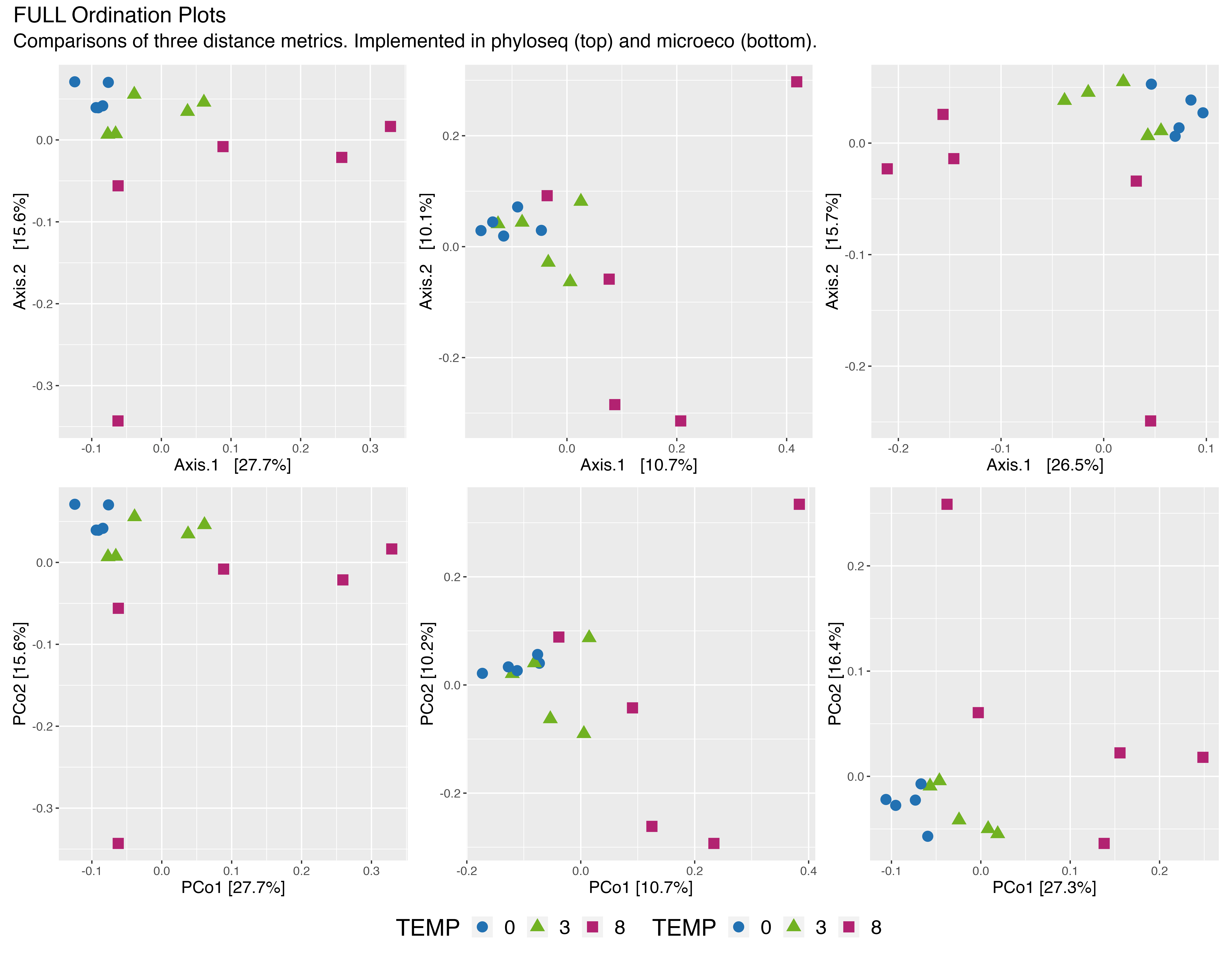(16S rRNA) Figure 1 | PCoA ordination plots of the FULL data set. Top = phyloseq, bottom = microeco; Left = Jensen-Shannon Divergence, middle = UniFrac (unwieghted), right = UniFrac (wieghted).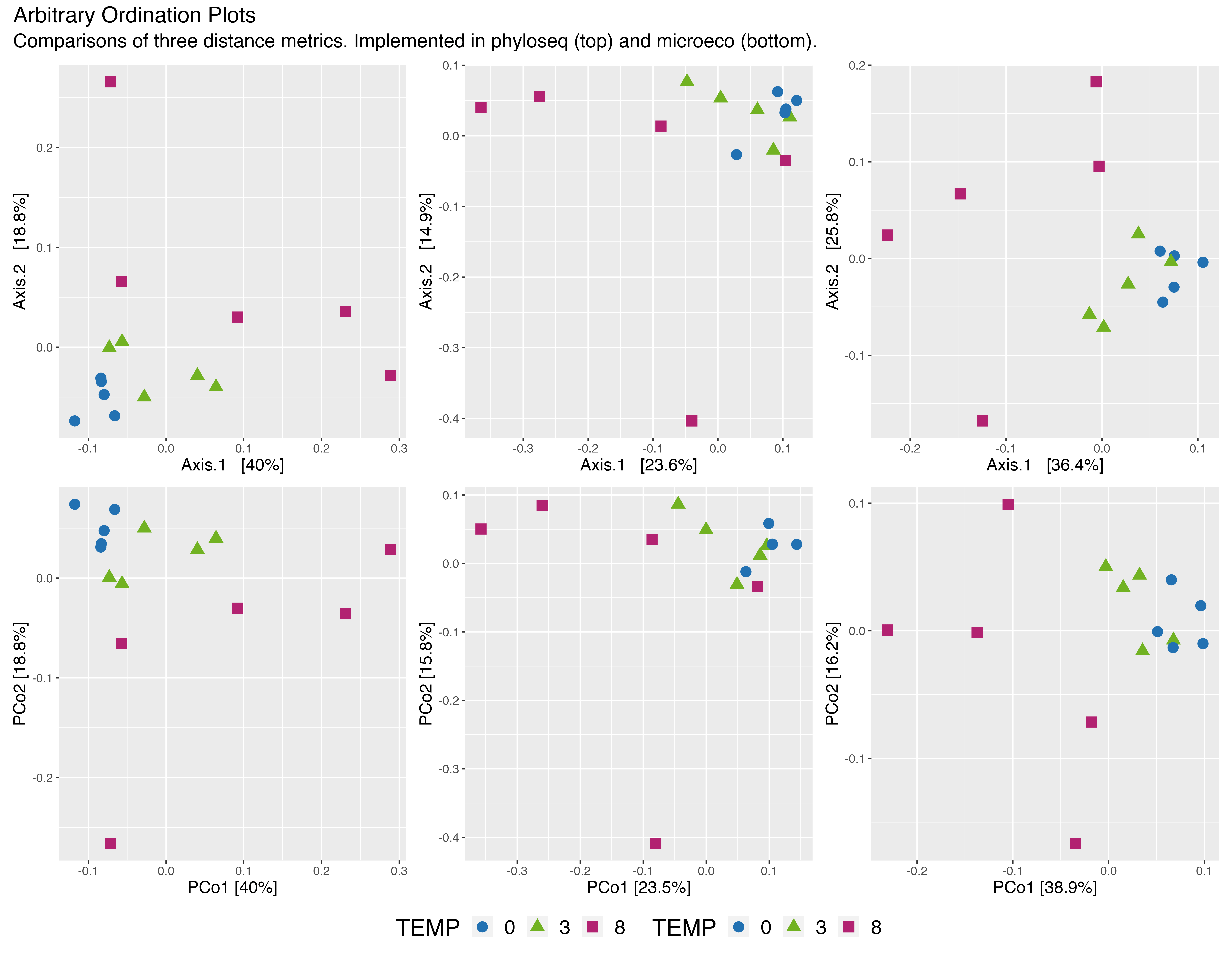(16S rRNA) Figure 2 | PCoA ordination plots of the Arbitrary filtered data set. Top = phyloseq, bottom = microeco; Left = Jensen-Shannon Divergence, middle = UniFrac (unwieghted), right = UniFrac (wieghted).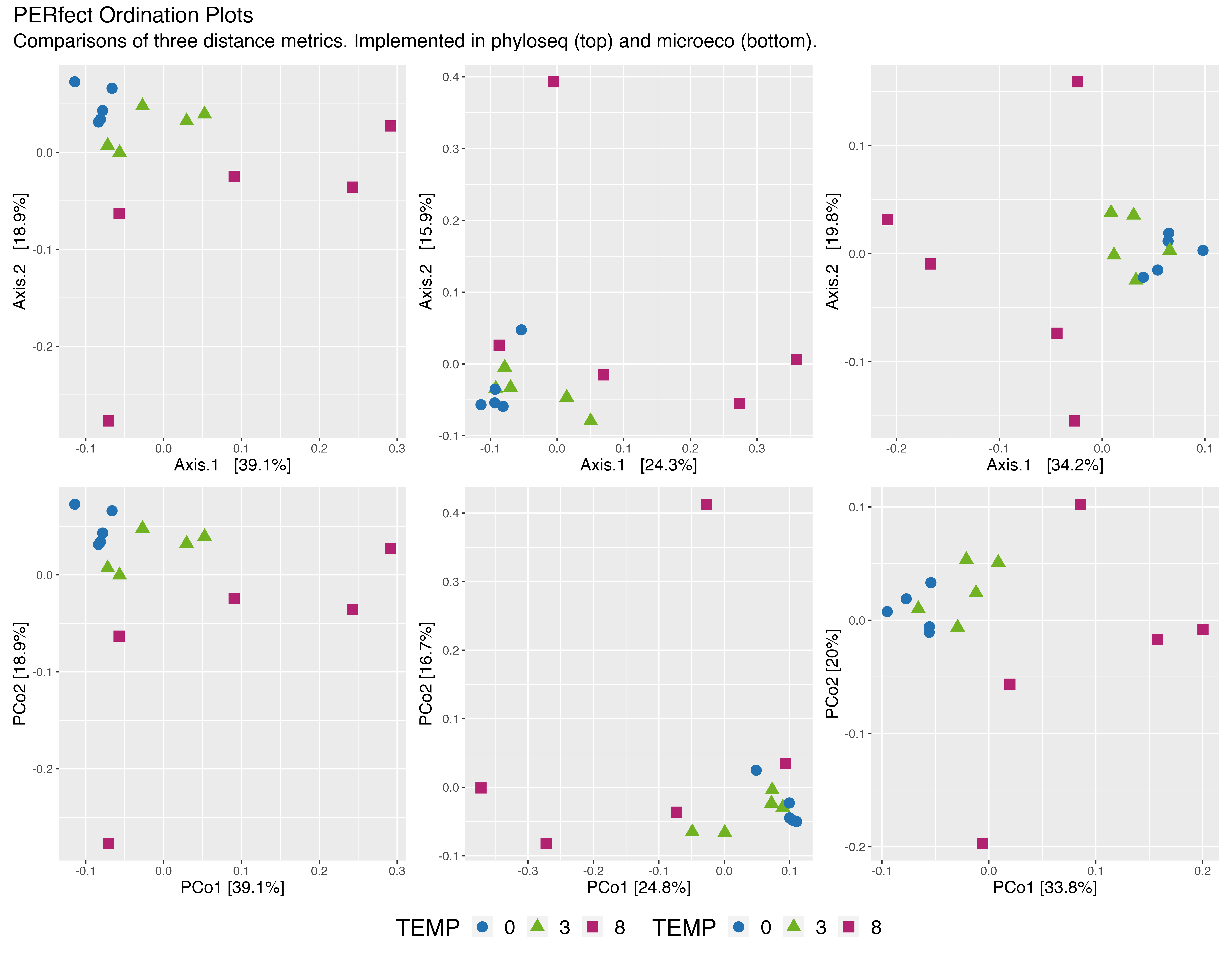(16S rRNA) Figure 3 | PCoA ordination plots of the PERfect filtered data set. Top = phyloseq, bottom = microeco; Left = Jensen-Shannon Divergence, middle = UniFrac (unwieghted), right = UniFrac (wieghted).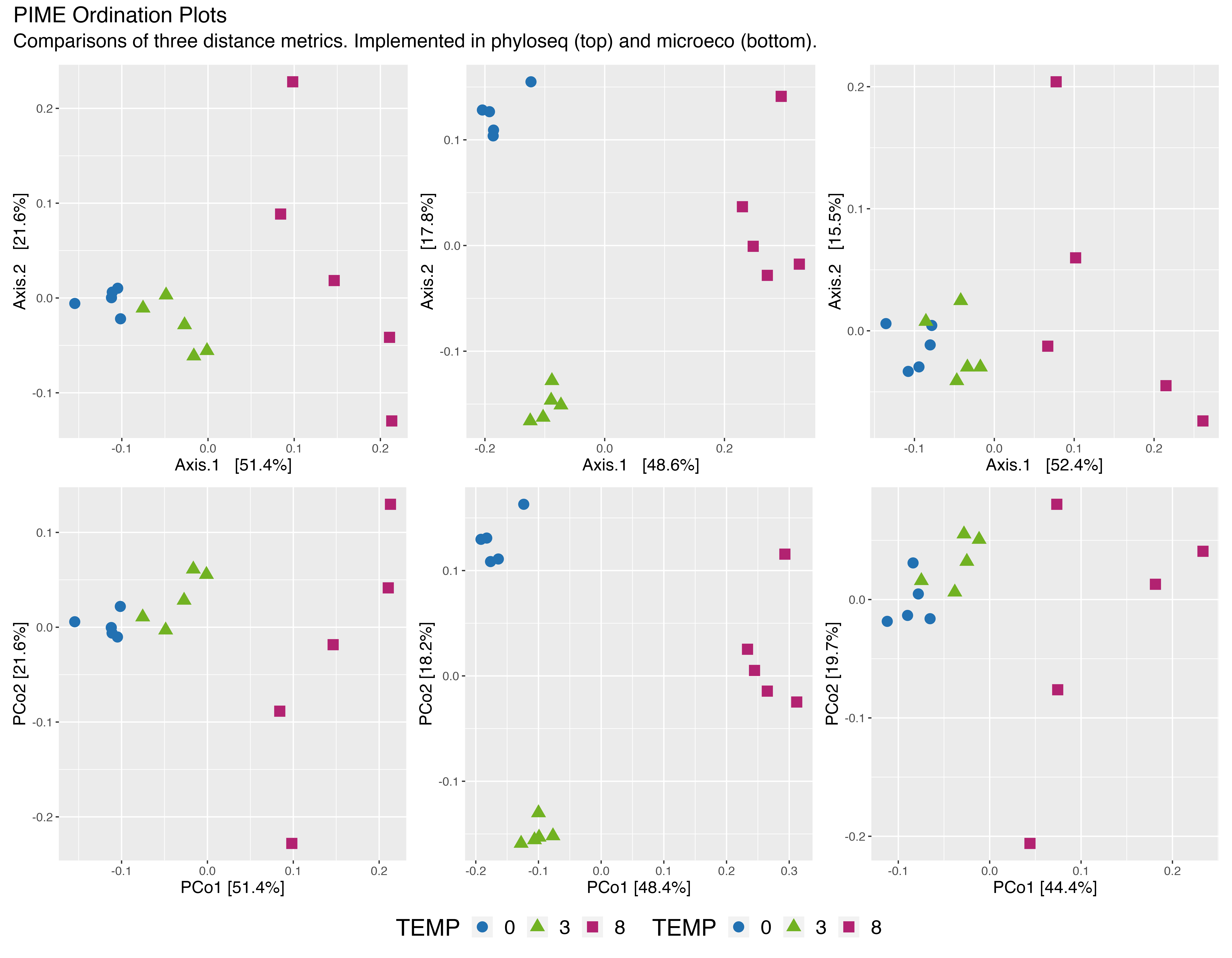(16S rRNA) Figure 4 | PCoA ordination plots of the PIME filtered data set. Top = phyloseq, bottom = microeco; Left = Jensen-Shannon Divergence, middle = UniFrac (unwieghted), right = UniFrac (wieghted).

## ITS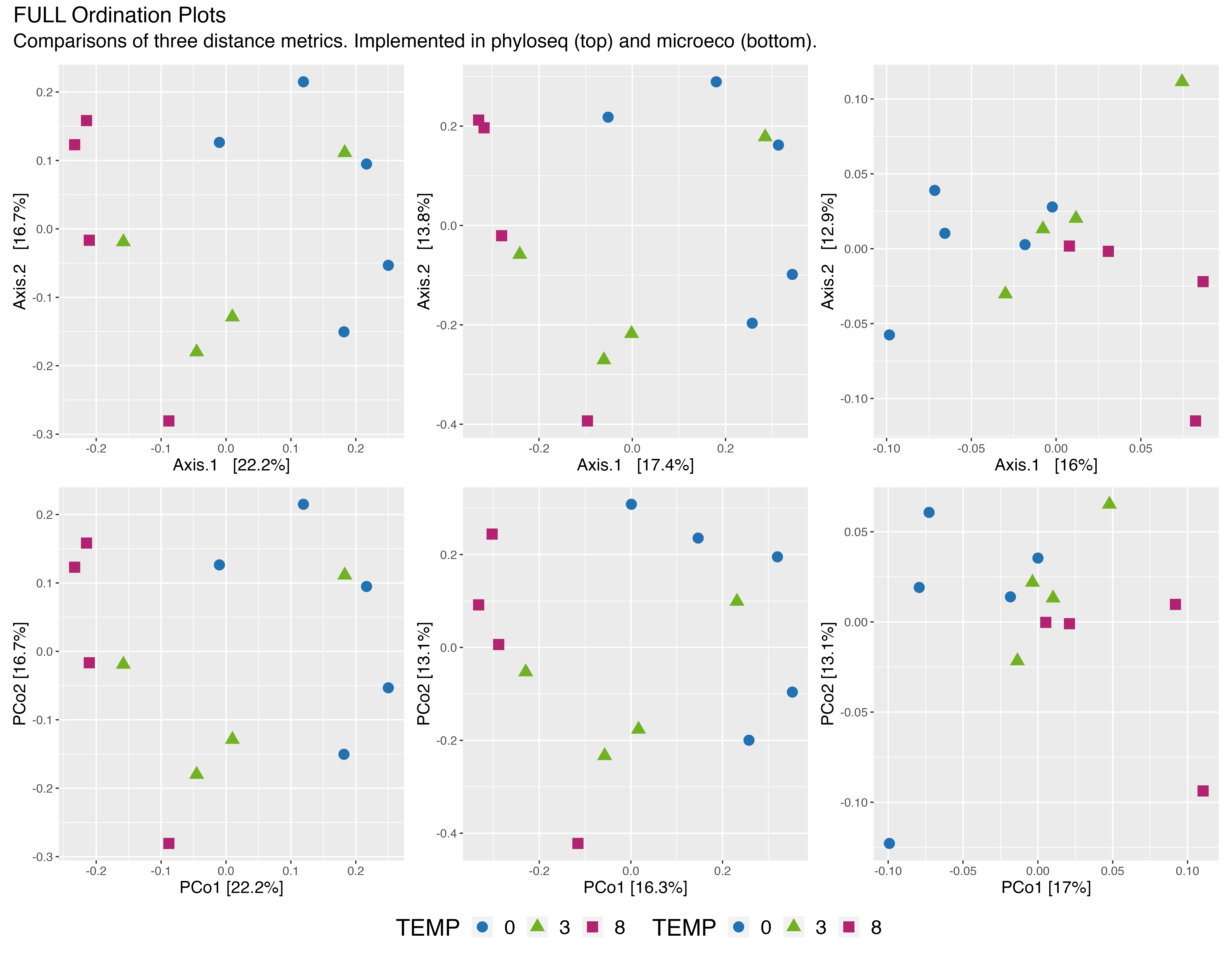(ITS) Figure 1 | PCoA ordination plots of the FULL data set. Top = phyloseq, bottom = microeco; Left = Jensen-Shannon Divergence, middle = Bray-Curtis dissimilarity, right = Gower distance.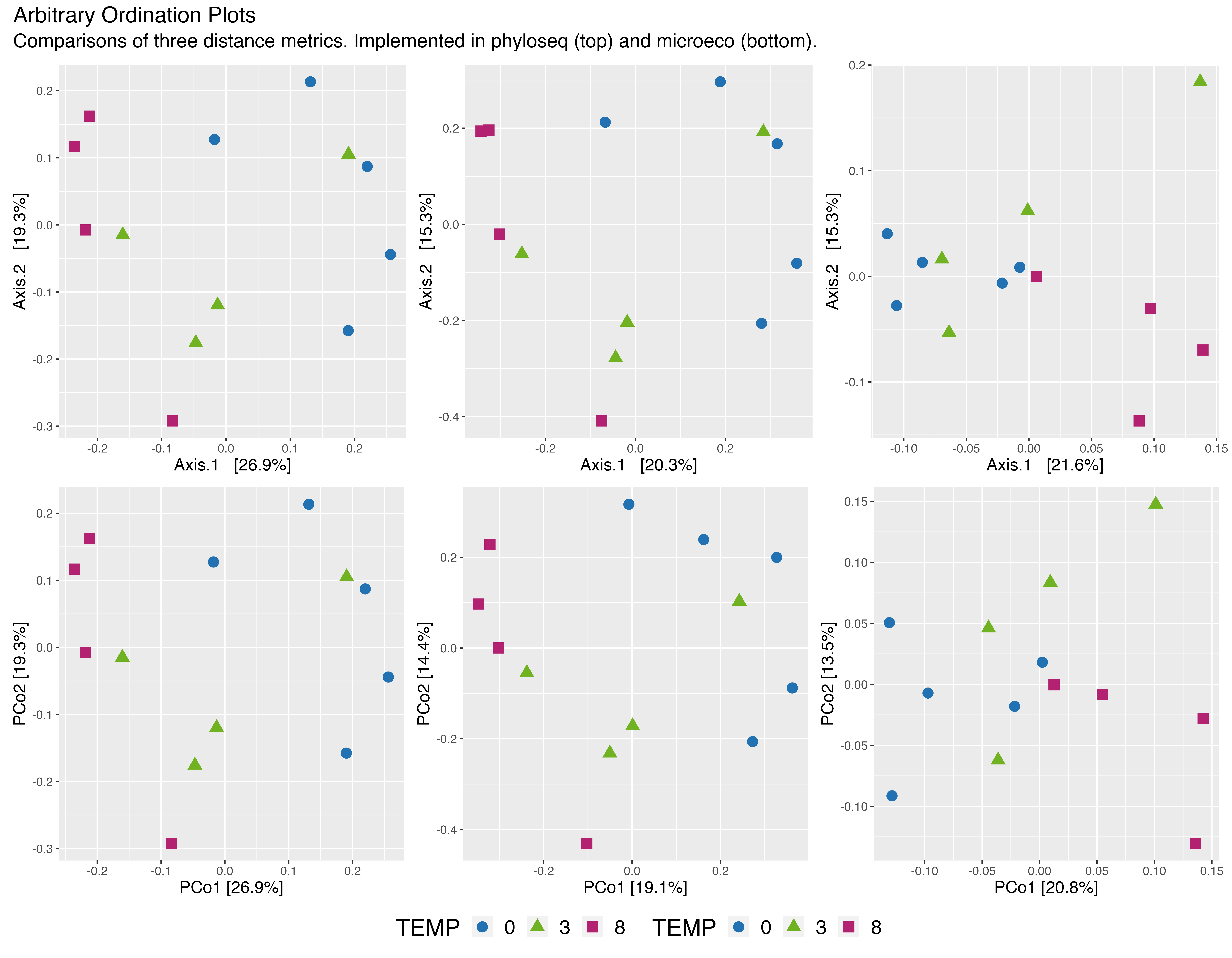(ITS) Figure 2 | PCoA ordination plots of the Arbitrary filtered data set. Top = phyloseq, bottom = microeco; Left = Jensen-Shannon Divergence, middle = Bray-Curtis dissimilarity, right = Gower distance.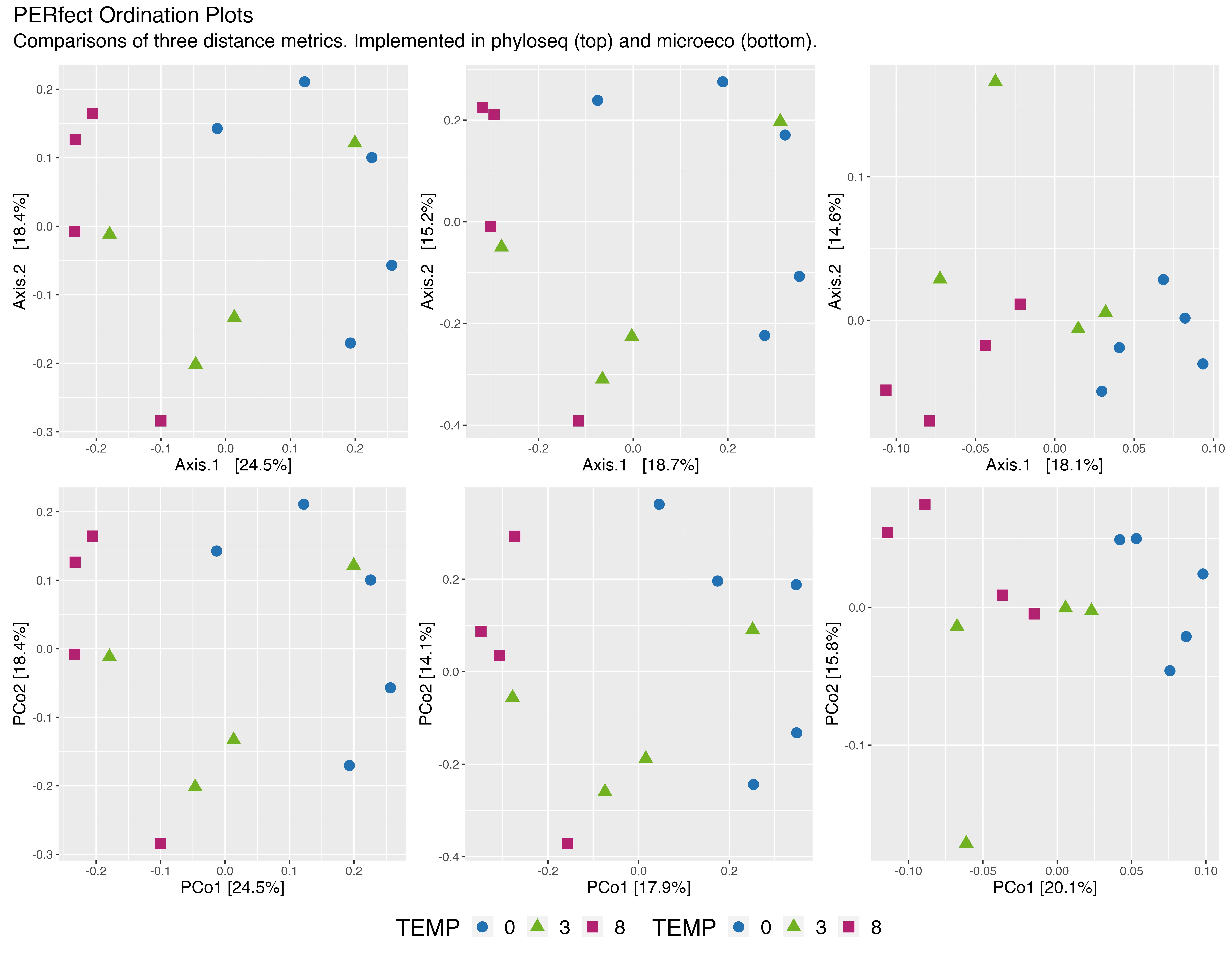(ITS) Figure 3 | PCoA ordination plots of the PERfect filtered data set. Top = phyloseq, bottom = microeco; Left = Jensen-Shannon Divergence, middle = Bray-Curtis dissimilarity, right = Gower distance.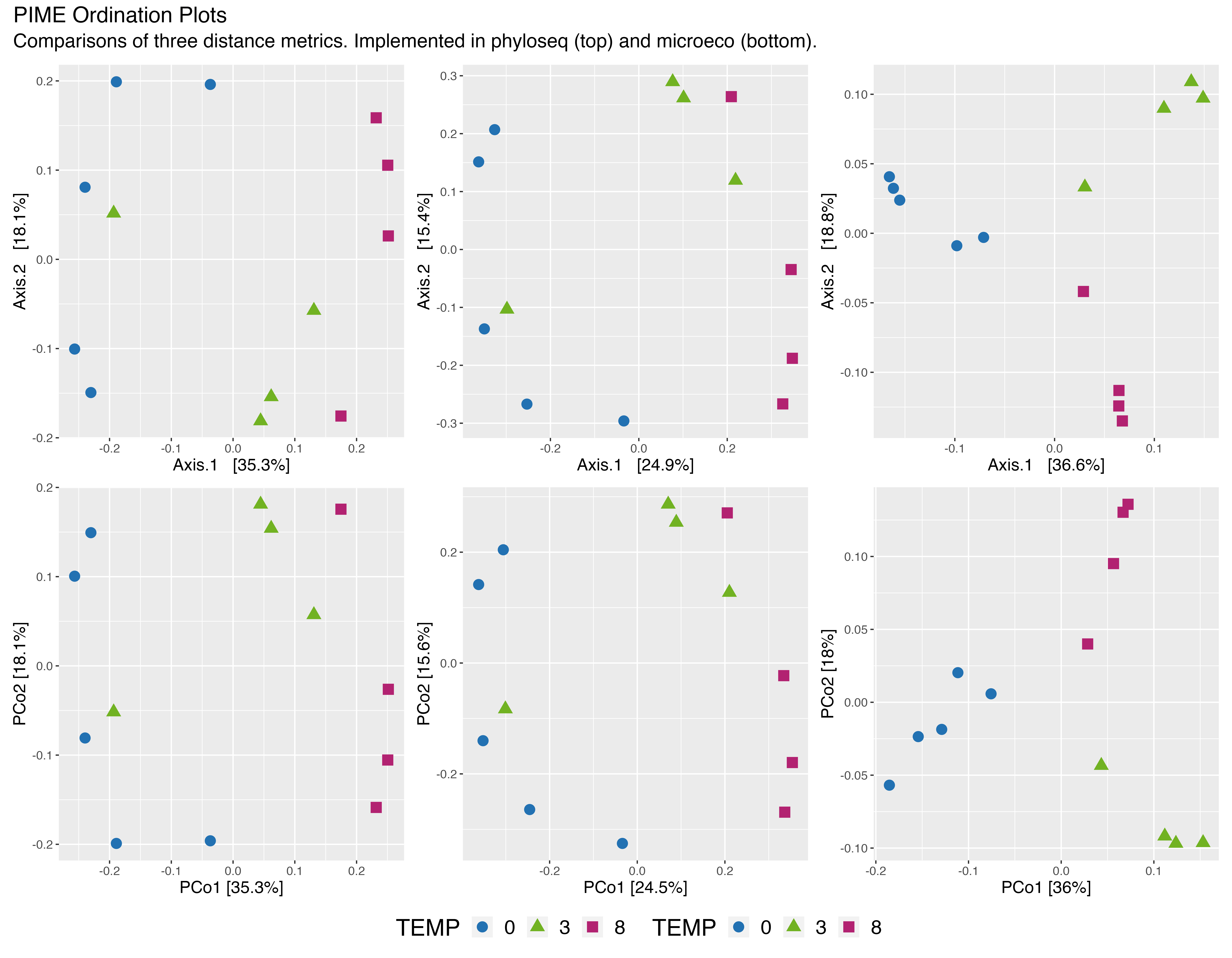(ITS) Figure 4 | PCoA ordination plots of the PIME filtered data set. Top = phyloseq, bottom = microeco; Left = Jensen-Shannon Divergence, middle = Bray-Curtis dissimilarity, right = Gower distance.

# Within & Between Group Distances

Above we calculated the sample distances within groups. Let’s see what those plots look like.

## 16S rRNA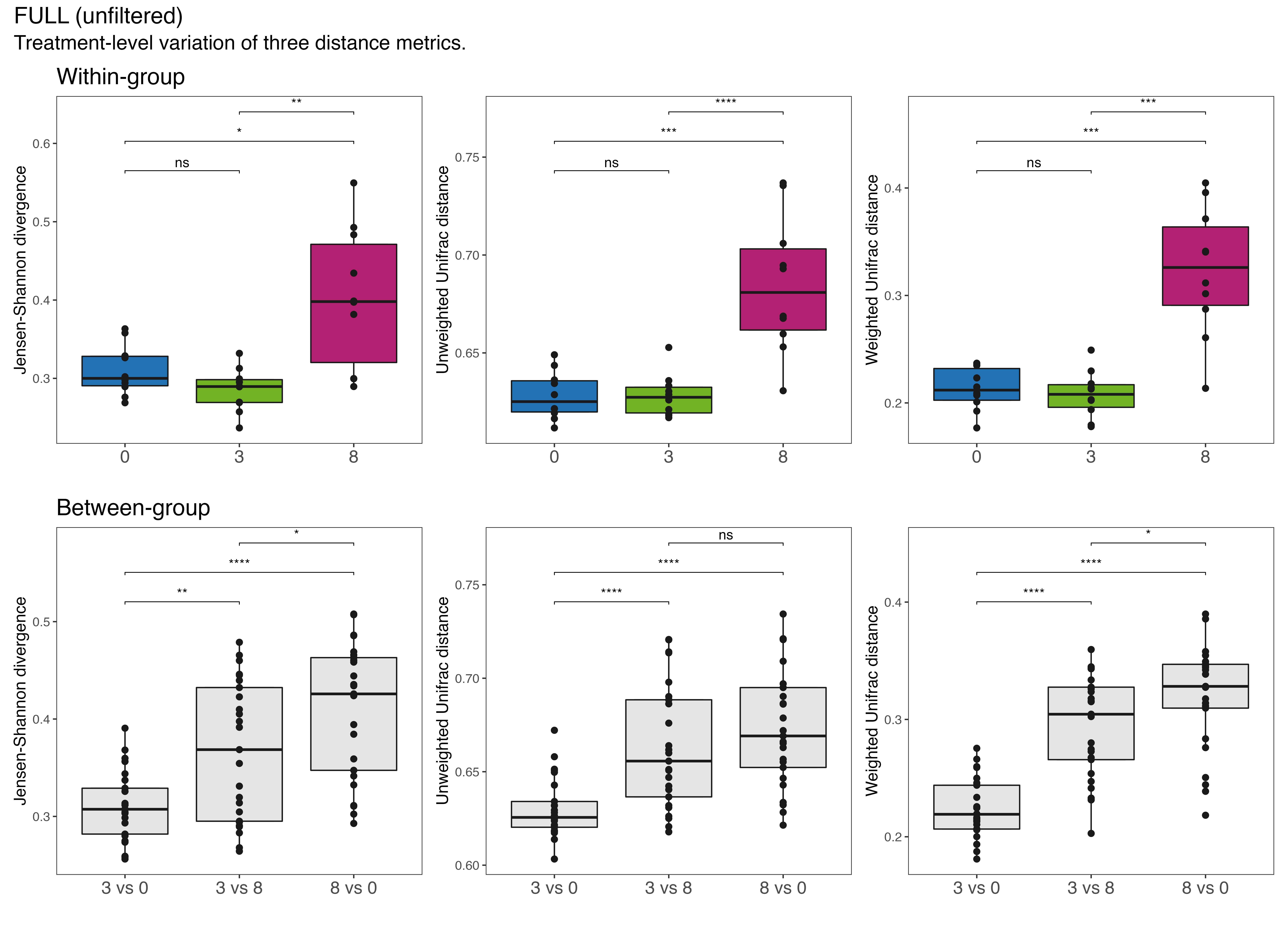(16S rRNA) Figure 5 | Within (top) and between (bottom) group variation plots of the FULL data set. Left = Jensen-Shannon Divergence, middle = UniFrac (unwieghted), right = UniFrac (wieghted). Significant differences denoted by asterisks (* p < 0.05, ** p < 0.01, *** p < 0.001, **** p < 0.0001). ns = not significant.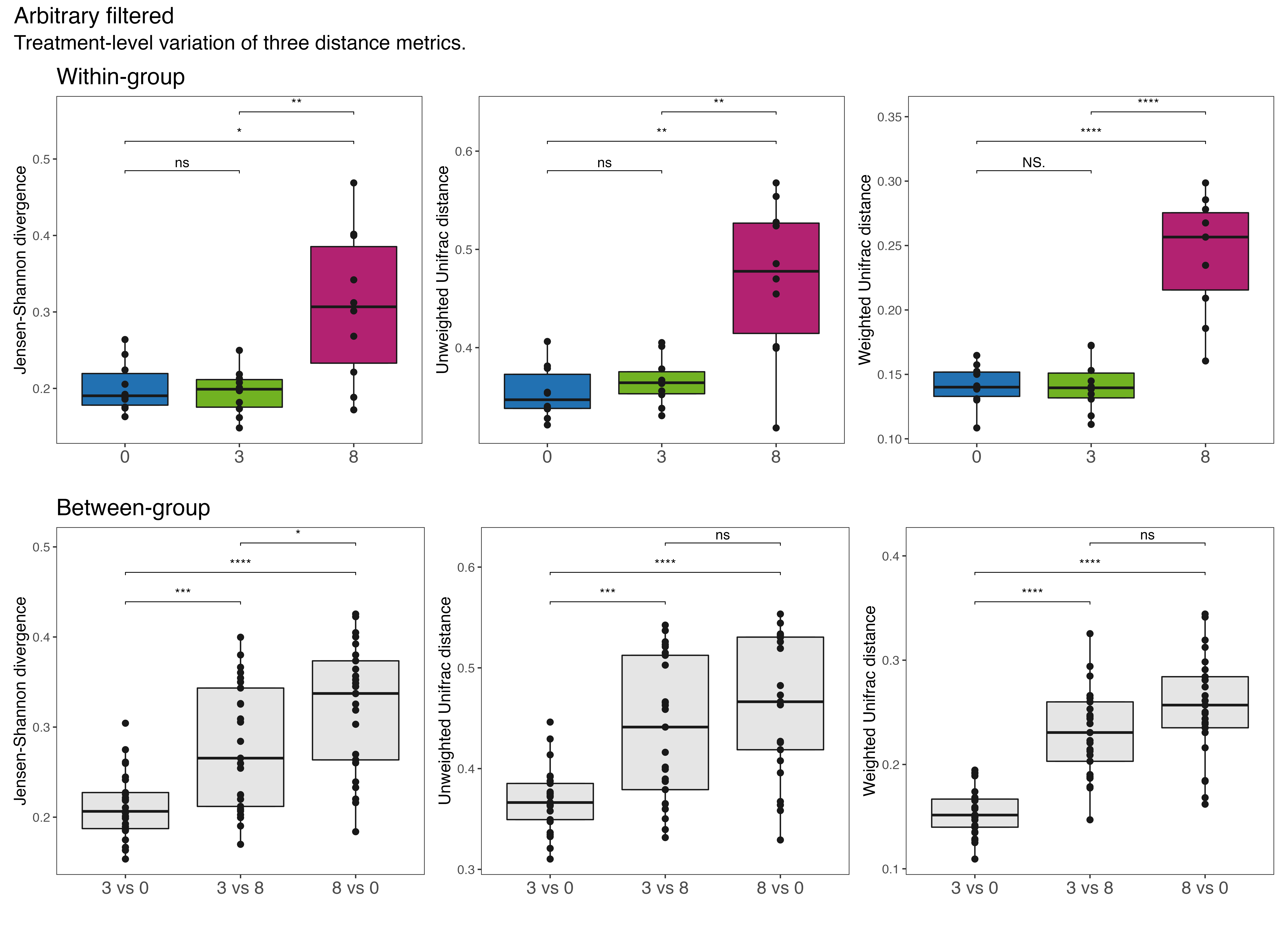(16S rRNA) Figure 6 | Within (top) and between (bottom) group variation plots of the Arbitrary filtered data set. Left = Jensen-Shannon Divergence, middle = UniFrac (unwieghted), right = UniFrac (wieghted). Significant differences denoted by asterisks (* p < 0.05, ** p < 0.01, *** p < 0.001, **** p < 0.0001). ns = not significant.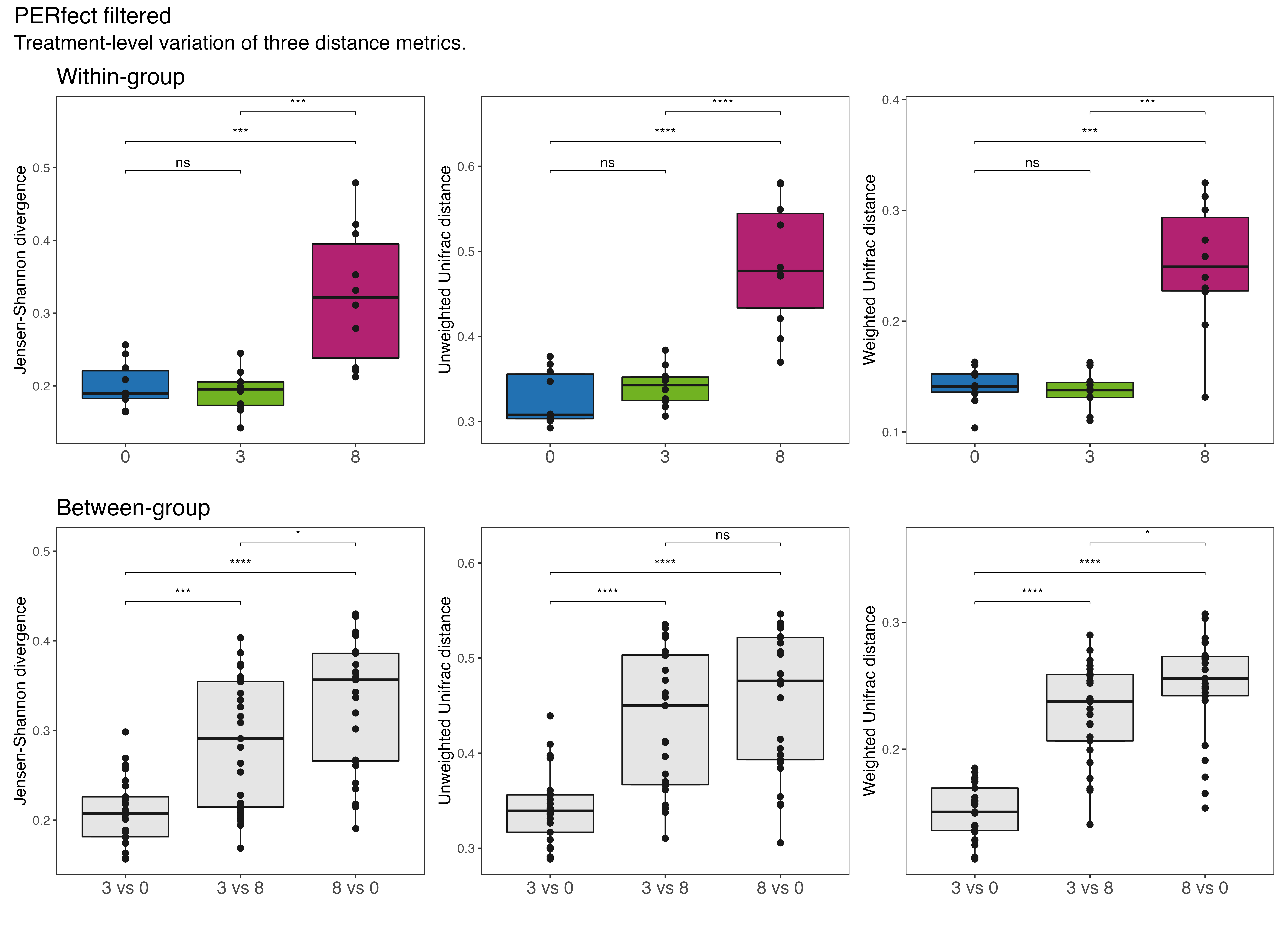(16S rRNA) Figure 7 | Within (top) and between (bottom) group variation plots of the PERfect filtered data set. Left = Jensen-Shannon Divergence, middle = UniFrac (unwieghted), right = UniFrac (wieghted). Significant differences denoted by asterisks (* p < 0.05, ** p < 0.01, *** p < 0.001, **** p < 0.0001). ns = not significant.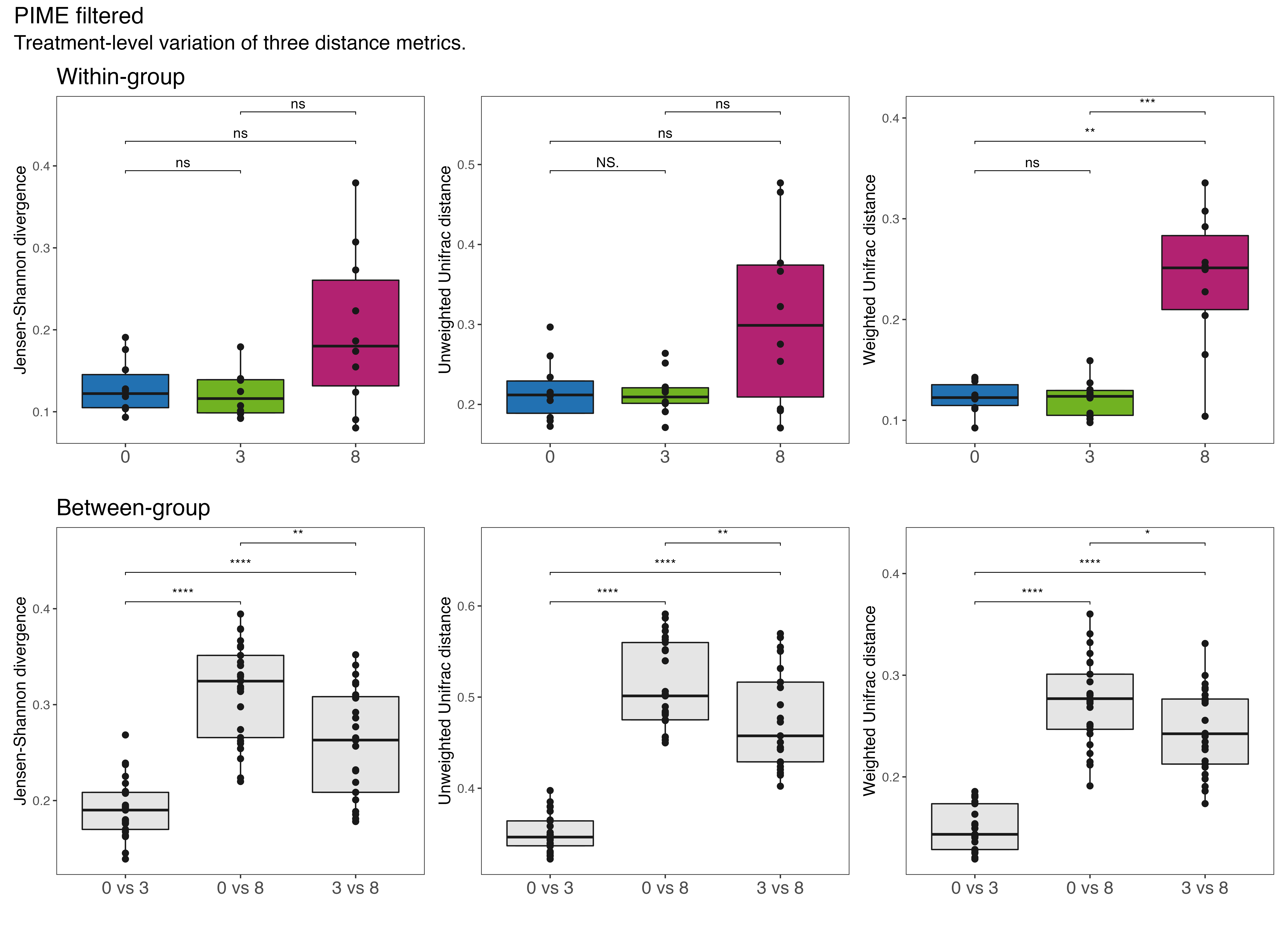(16S rRNA) Figure 8 | Within (top) and between (bottom) group variation plots of the PIME filtered data set. Left = Jensen-Shannon Divergence, middle = UniFrac (unwieghted), right = UniFrac (wieghted). Significant differences denoted by asterisks (* p < 0.05, ** p < 0.01, *** p < 0.001, **** p < 0.0001). ns = not significant.

## ITS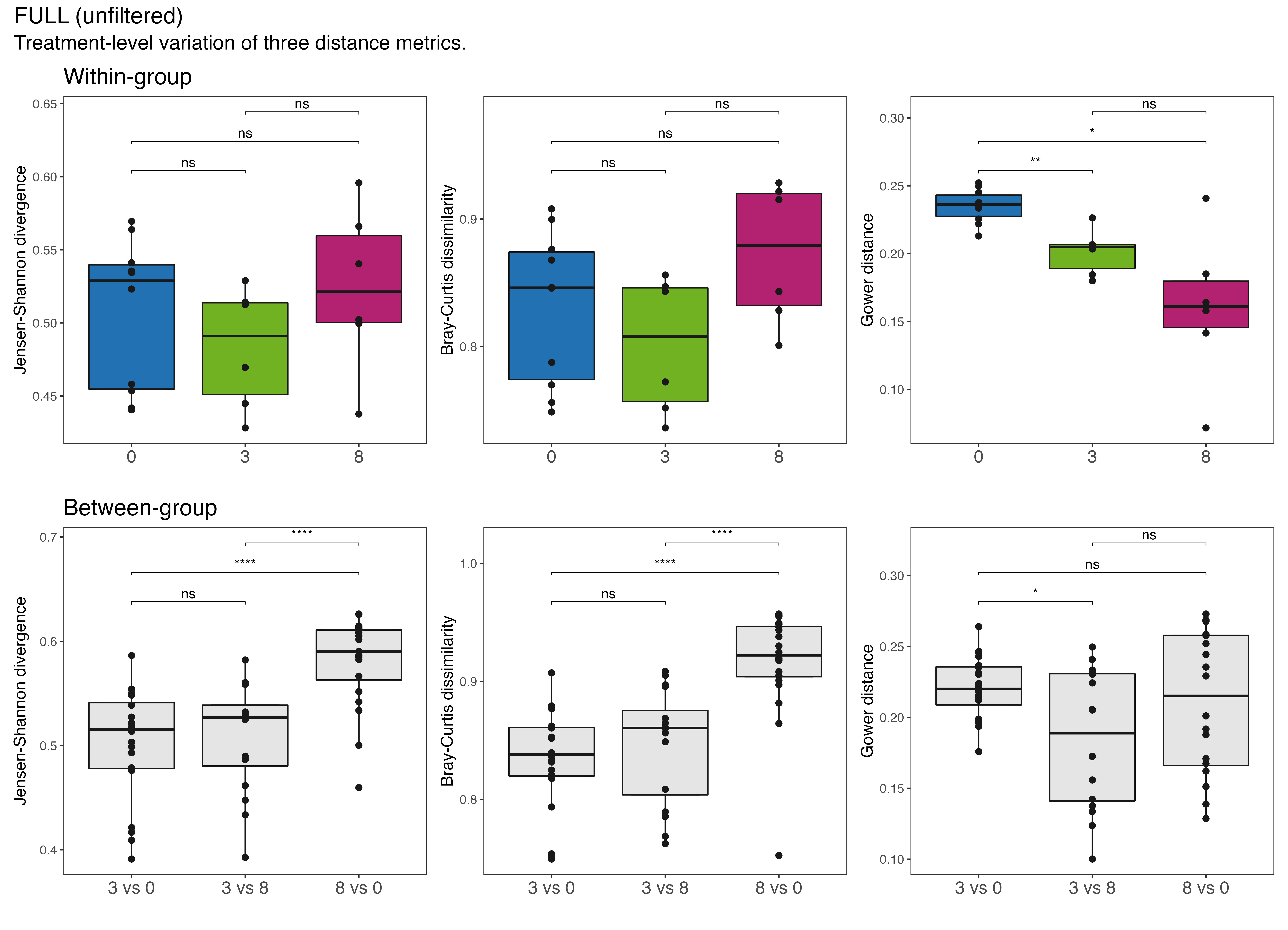(ITS) Figure 5 | Within (top) and between (bottom) group variation plots of the FULL data set. Left = Jensen-Shannon Divergence, middle = Bray-Curtis dissimilarity, right = Gower distance. Significant differences denoted by asterisks (* p < 0.05, ** p < 0.01, *** p < 0.001, **** p < 0.0001). ns = not significant.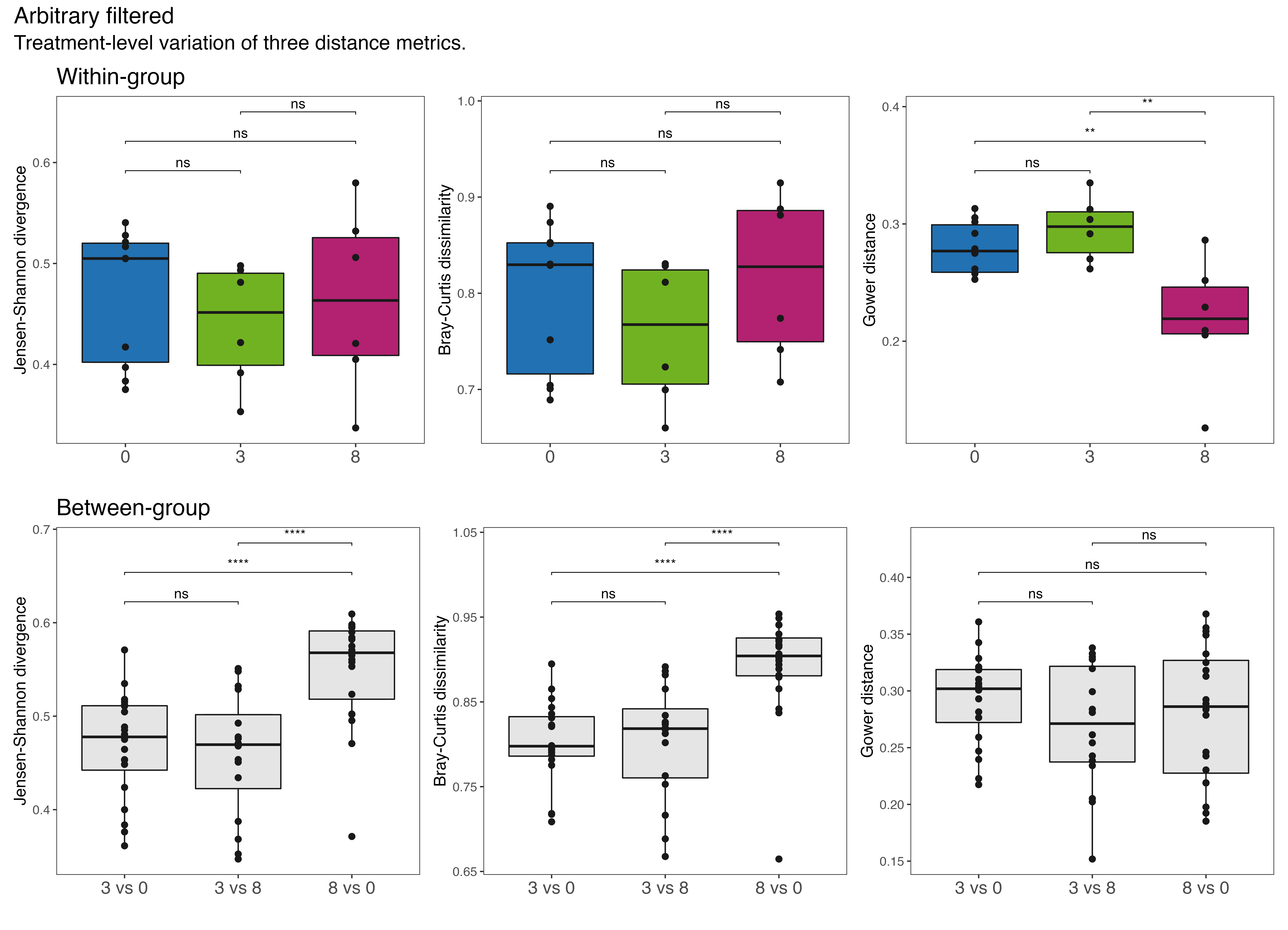(ITS) Figure 6 | Within (top) and between (bottom) group variation plots of the Arbitrary filtered data set. Left = Jensen-Shannon Divergence, middle = Bray-Curtis dissimilarity, right = Gower distance. Significant differences denoted by asterisks (* p < 0.05, ** p < 0.01, *** p < 0.001, **** p < 0.0001). ns = not significant.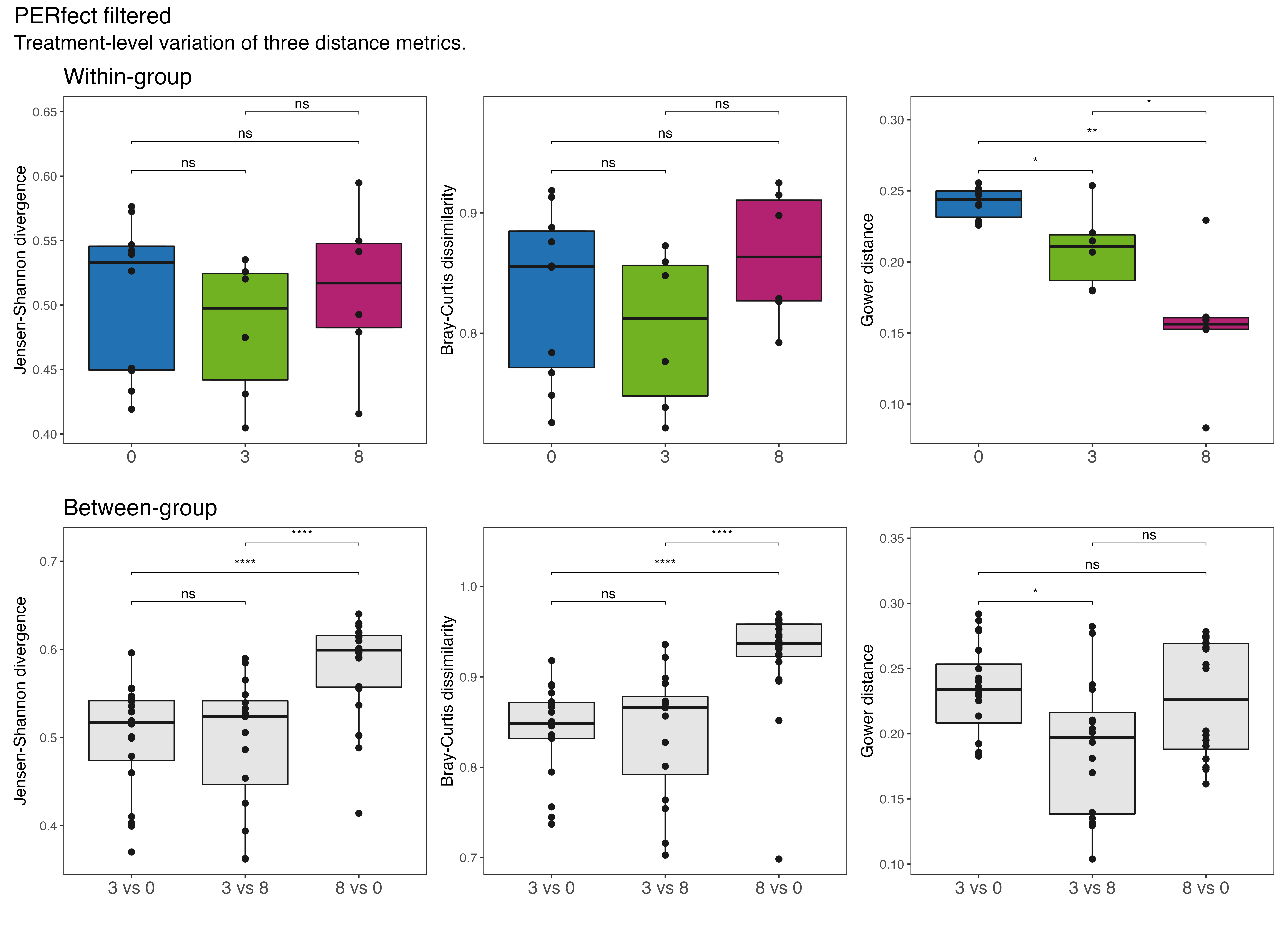(ITS) Figure 7 | Within (top) and between (bottom) group variation plots of the PERfect filtered data set. Left = Jensen-Shannon Divergence, middle = Bray-Curtis dissimilarity, right = Gower distance. Significant differences denoted by asterisks (* p < 0.05, ** p < 0.01, *** p < 0.001, **** p < 0.0001). ns = not significant.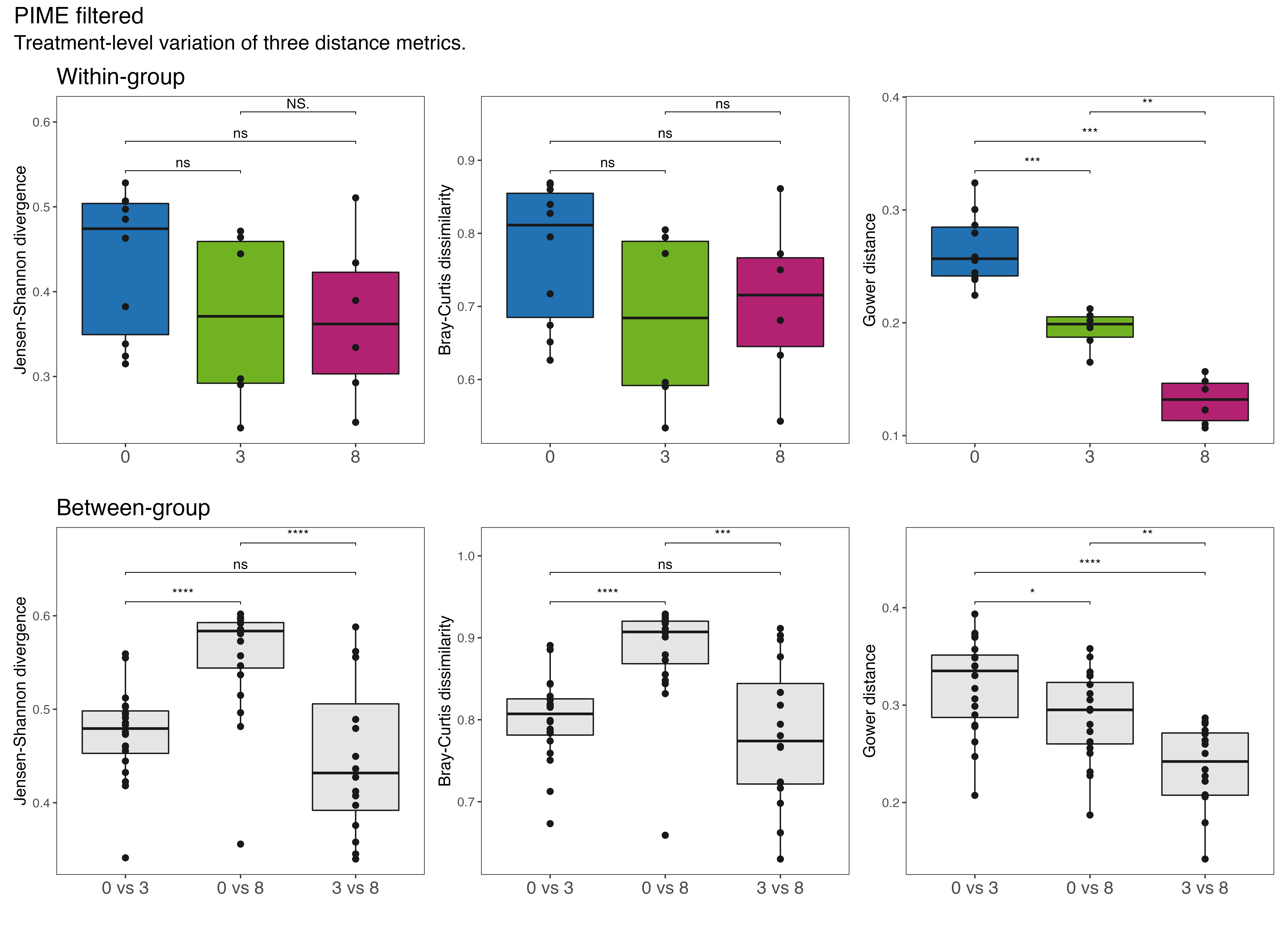(ITS) Figure 8 | Within (top) and between (bottom) group variation plots of the PIME filtered data set. Left = Jensen-Shannon Divergence, middle = Bray-Curtis dissimilarity, right = Gower distance. Significant differences denoted by asterisks (* p < 0.05, ** p < 0.01, *** p < 0.001, **** p < 0.0001). ns = not significant.

# Workflow Output

## Source Code

`` "2022-06-29 07:27:33 EST"``